• 机器学习项目
千次阅读
2021-12-01 11:34:39

机器学习听起来很像是：平板电脑、计算机等各种形式的设备根据编程和大量数据来学习一些东西。它看起来像一个未来主义概念，而实际上每天都在我们的现实生活中被广泛应用，语音识别就是一个很好的例子。如同Siri 和 Alexa 这样的虚拟助手就是通过机器学习技术来实现语音提醒、回答问题、执行命令等功能。

随着机器学习的激增，越来越多的专业人士选择从事机器学习工程师的职业。而成为机器学习工程师最好的入门方法之一就是亲自动手开发一个项目，网上有许多免费资源课程。

今天我们就来简单聊一聊10个机器学习项目，先睹为快，上列表！

目录

1. 使用 Movielens 数据集推荐电影
2. TensorFlow
3. 沃尔玛的销售预测
4. 股价预测
5. 使用智能手机识别人类活动
6. 葡萄酒质量预测
7. 乳腺癌预测
8. 虹膜分类
10. 将手写文档数字化

接下来我们逐一介绍

## 1. 使用Movielens 数据集推荐电影

流媒体时代，几乎每个人都会在媒体平台上观看自己喜欢的电影或电视节目。虽然有时我们自己也会苦于剧荒，不知道一剧终结后下一部何去何从，但是“过分聪明”的平台则通常会根据我们的历史记录和偏好来做出响应推荐。而这一过程就是通过机器学习来完成的。对于初学者来说，这是一个有趣且简单的项目。新程序员可以通过使用Python 或 R语言以及来自Movielens数据集的数据进行编码来练习。Movielens 数据集由 6,000 多个用户生成，目前包含 3,900 部超过百万评级的电影。

## 2. TensorFlow

这个开源人工智能库是帮助初学者提高机器学习技能的神器。借助TensorFlow，学员可以使用这个智能库来创建数据流图、使用 Java 的项目以及一系列应用程序，其中还包括用于 Java 的 API。

## 3. 沃尔玛的销售预测

虽然我们可能无法对未来的销售额做出精准预测，但使用机器学习却能为我们指明销售方向。例如，沃尔玛为45 个网点的 98 种产品提供数据集，然后开发人员可以访问按地点和部门划分的每周销售信息。此范围项目的目的在于通过优化渠道和库存计划从而做出更好的数据驱动决策。

## 4. 股价预测

与销售预测类似，股价预测基于过去价格、波动率指数和基本面指标的数据集。初学者可以从这样的项目开始，然后使用股票市场数据集来创建未来几个月的预测。通过这种方法我们可以更好地熟悉怎样通过大量数据集来创建预测。从 Quantopian 或Quandl下载股票市场数据集，开始练习吧！

## 5. 使用智能手机识别人类活动

如今，移动设备都能自动检测出我们在哪些时间进行了哪些特定活动，比如跑步、骑自行车、散步等。这些都是机器学习在发挥作用。想要练习此类项目，机器学习工程师新手需要引用一个数据集，其中包含通过配备惯性传感器的移动设备收集的少数人（当然越多越好）的健身活动记录。然后，学员可以构建分类模型，以便准确预测未来的活动。这也可以帮助我们了解如何解决多分类问题。

## 6. 葡萄酒质量预测

购买新的或不熟悉的葡萄酒可能有些冒险，万一不对口，也会成为“人生一憾事”。除非你是一位通晓各种判定因素（如年份和价格）的专家，否则不能准确判定出某款葡萄酒是否具有高品质。葡萄酒质量数据集中就包含这样的细节，它可以帮助我们通过机器学习来预测出葡萄酒的质量，这个项目是不是很有趣？此外，通过这个项目，ML 初学者可以获得数据可视化、数据探索、回归模型和 R 编程方面的经验。

## 7. 乳腺癌预测

这个机器学习项目使用的数据集可以帮我们确定乳腺肿瘤可能是恶性还是良性。项目会综合考虑各种因素，包括肿块的厚度、裸核的数量和有丝分裂等。这也是机器学习专业新手士练习R 编程的绝佳机会。

## 8. 虹膜分类

鸢尾花数据集非常有名，也是适合初学者的最古老、最简单的机器学习项目之一。通过这个项目，学习者必须弄清楚处理数值和数据的基础知识。数据点包括按长度和宽度划分的萼片和花瓣的大小。使用机器学习，一个成功的项目将鸢尾花分为三个物种之一。

浏览推文时，能快速筛选出包含特定关键词和信息的文章是一种超级棒的体验。想要实现这种功能，只需要练习一个初学者级别的机器学习项目。程序员创建一种算法，该算法通过自然语言处理器运行抓取的推文来确定哪些更可能匹配特定主题、谈论某些个人等等，是不是so easy?

## 10. 将手写文档数字化

这种类型的项目是练习深度学习和神经网络（机器学习中图像识别的基本要素）的绝佳方法。初学者还可以学习如何将像素数据转换为图像，以及如何使用逻辑回归和 MNIST 数据集。

## 获得机器学习认证

如果你的目标是锁定一门从基础知识到机器学习算法开发和无监督学习等高级技术都包含在内的系统课程，那么建议考虑一下Simplilearn 的机器学习认证培训课程。它为初学者提供了一系列机器学习项目，包括超过 25 个机器学习练习。该课程还包括 44 小时的讲师指导培训和机器学习专家的指导课程。

发现一门好课程，义无反顾地为自己做一次投资，不久后会庆幸自己此时的决定有多明智。大数据 人工智能
更多相关内容
• 涵盖面向初学者，中级专家和专家的23种机器学习项目创意，以获取有关该增长技术的真实经验。这些机器学习项目构想将帮助你了解在职业生涯中取得成功、和当下就业所需的所有实践。 通过项目学习是你短期内能做的最好...涵盖面向初学者，中级专家和专家的23种机器学习项目创意，以获取有关该增长技术的真实经验。这些机器学习项目构想将帮助你了解在职业生涯中取得成功、和当下就业所需的所有实践。

通过项目学习是你短期内能做的最好投资，这些项目构想使你能够快速发展和增强机器学习技能。语言上，这些机器学习项目可以用Python，R或任何其他工具开发。

目录

面向初学者的机器学习项目

1.鸢尾花分类项目

2. Emojify –使用Python创建自己的表情符号

3.使用机器学习进行贷款预测

4.住房价格预测项目

5. MNIST数字分类机器学习项目

6.使用机器学习预测股价

7.泰坦尼克号生存计划

8.葡萄酒质量检测项目

9.假新闻检测项目

中级机器学习项目

1.音乐流派分类机器学习项目

2.比特币价格预测器项目

3. Uber数据分析项目

4.人格预测项目

5. Xbox游戏预测项目

6.信用卡欺诈检测项目

7.芭比与大脑项目

8.使用机器学习进行客户细分

高级机器学习项目

1.使用机器学习进行情感分析

2.安然调查项目

3.语音情感识别机器学习项目

4.抓非法捕鱼项目

5.使用协同过滤的在线杂货推荐

6.使用机器学习的电影推荐系统

7.车牌自动识别系统

## 面向初学者的机器学习项目

在本部分中，我们列出了针对初学者/初学者的顶级机器学习项目，如果你已经从事基础机器学习项目，请跳至下一部分：中级机器学习项目

### 1.鸢尾花分类项目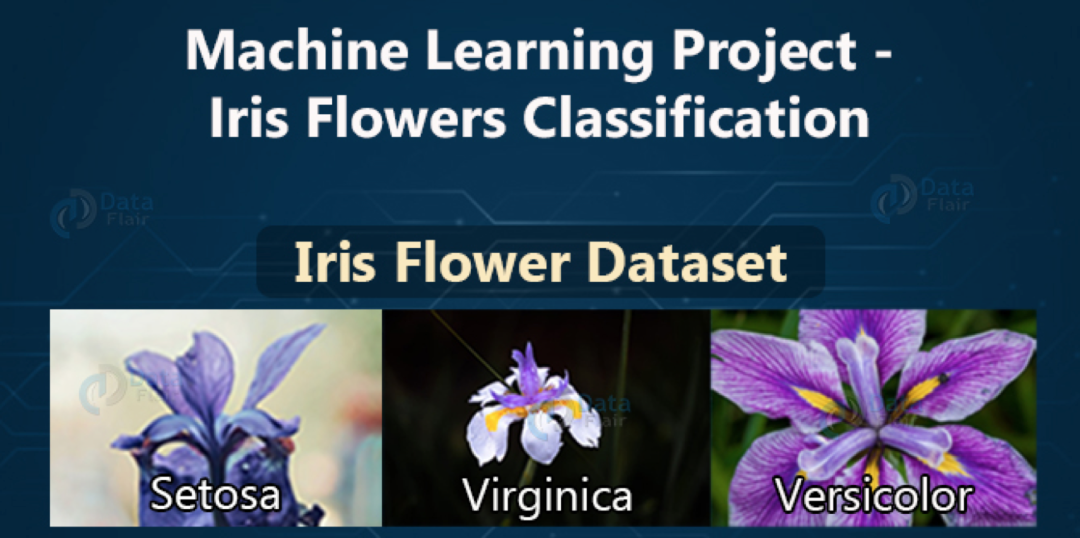项目构想鸢尾花有不同的种类，你可以根据花瓣和萼片的长度来区分它们。这是机器学习初学者预测新鸢尾花种类的基础项目。

数据集：鸢尾花分类数据集

https://archive.ics.uci.edu/ml/datasets/Iris

### 2. Emojify –使用Python创建自己的表情符号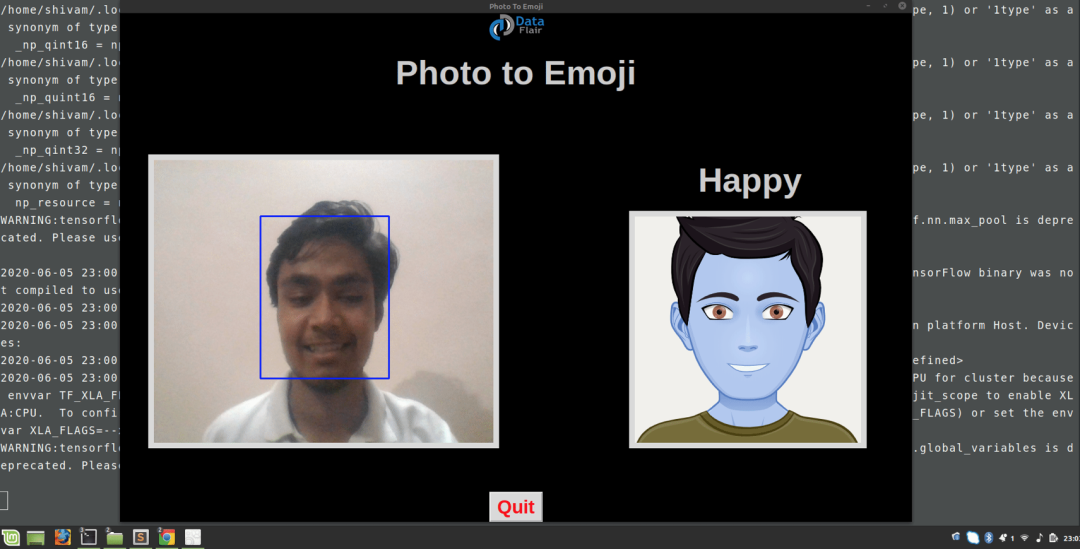项目构想：该机器学习项目的目标是对人的面部表情进行分类并将其映射为表情符号。我们将建立一个卷积神经网络来识别面部表情。然后，我们将使用相应的表情符号或头像来映射这些情感。

源代码：Emojify项目

https://data-flair.training/blogs/create-emoji-with-deep-learning/

### 3.使用机器学习进行贷款预测

项目构想：该ML项目背后的构想是建立一个模型，该模型将对用户可以贷款多少进行分类。它基于用户的婚姻状况，教育程度，受抚养人数和就业情况。我们可以为此项目建立一个线性模型。

数据集：贷款预测数据集

https://www.kaggle.com/altruistdelhite04/loan-prediction-problem-dataset

### 4.住房价格预测项目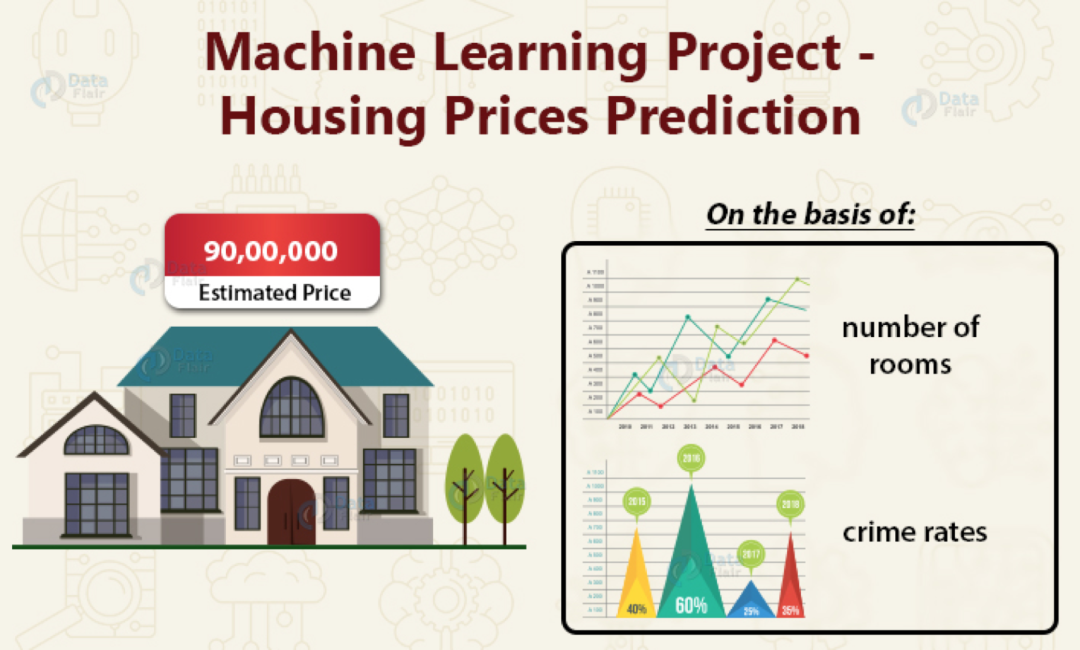项目构想：数据集包含波士顿剩余区域的房价。房子的费用根据犯罪率，房间数量等各种因素而变化。对于初学者来说，这是一个很好的ML项目，它可以根据新数据预测价格。

数据集：房屋价格预测数据集

https://www.cs.toronto.edu/~delve/data/boston/bostonDetail.html

### 5. MNIST数字分类机器学习项目

项目构想：MNIST数字分类python项目使机器能够识别手写数字。该项目对于计算机视觉可能非常有用。在这里，我们将使用MNIST数据集使用卷积神经网络训练模型。

数据集：MNIST数字识别数据集

源代码：手写数字识别项目

https://data-flair.training/blogs/python-deep-learning-project-handwritten-digit-recognition/

### 6.使用机器学习预测股价

项目构想：有许多可用于股票市场价格的数据集。这个机器学习初学者的项目旨在根据上一年的数据预测股票市场的未来价格。

数据集：股票价格预测数据集

https://www.kaggle.com/c/two-sigma-financial-news/data

源代码：股票价格预测项目

https://data-flair.training/blogs/stock-price-prediction-machine-learning-project-in-python/

### 7.泰坦尼克号生存计划

项目构想：这将是一个有趣的项目，因为我们将预测某人是否会在泰坦尼克号飞船中幸存下来。对于这个初学者的项目，我们将使用泰坦尼克号数据集，其中包含幸存者和在泰坦尼克号飞船中死亡的人的真实数据。

数据集：《泰坦尼克号生存》数据集

https://www.kaggle.com/c/titanic

### 8.葡萄酒质量检测项目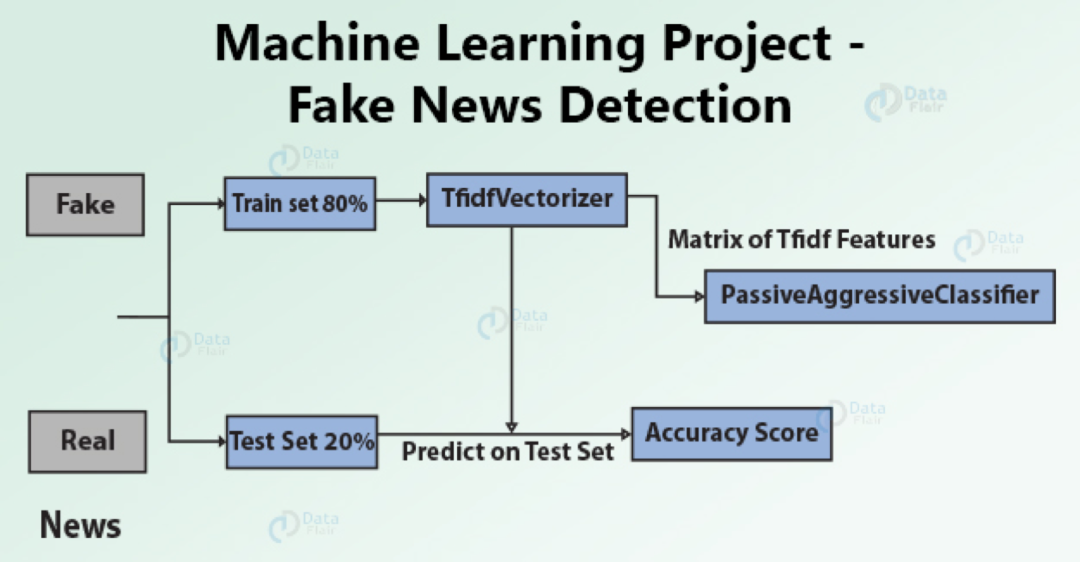项目构想：在该项目中，我们可以构建一个界面来预测红酒的质量。它将使用葡萄酒的化学信息，并基于机器学习模型，它将为我们提供葡萄酒质量的结果。

数据集：葡萄酒质量数据集

https://archive.ics.uci.edu/ml/datasets/wine+quality

### 9.假新闻检测项目

项目构想：虚假新闻像野火一样传播，这在这个时代是一个大问题。我们可以学习如何将假新闻与真实新闻区分开。我们可以使用监督学习来实现这样的模型。

数据集：检测虚假新闻数据集

源代码：假新闻检测项目

## 中级机器学习项目

### 1.音乐流派分类机器学习项目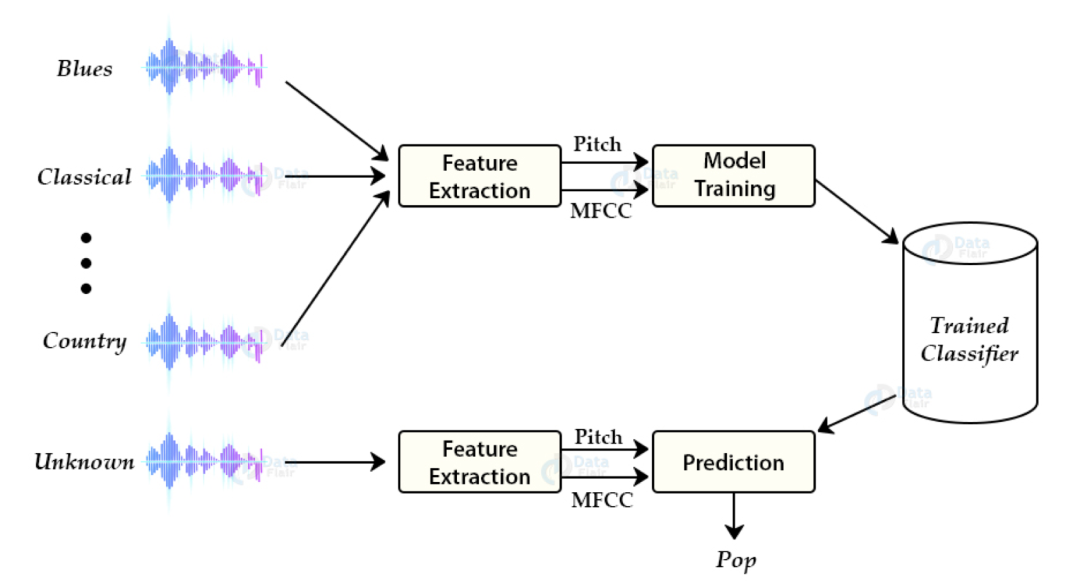项目构想：该python机器学习项目的思想是开发一个机器学习项目，并自动对音频中的不同音乐流派进行分类。我们需要使用它们的频域和时域低级特征对这些音频文件进行分类。

源代码：音乐流派分类项目

https://data-flair.training/blogs/python-project-music-genre-classification/

### 2.比特币价格预测器项目

项目构想：比特币价格预测器是一个有用的项目。区块链技术正在发展，并且有许多数字货币在上升。该项目将帮助你使用以前的数据预测比特币的价格。

数据集：比特币价格预测数据集

https://www.kaggle.com/team-ai/bitcoin-price-prediction/version/1

### 3. Uber数据分析项目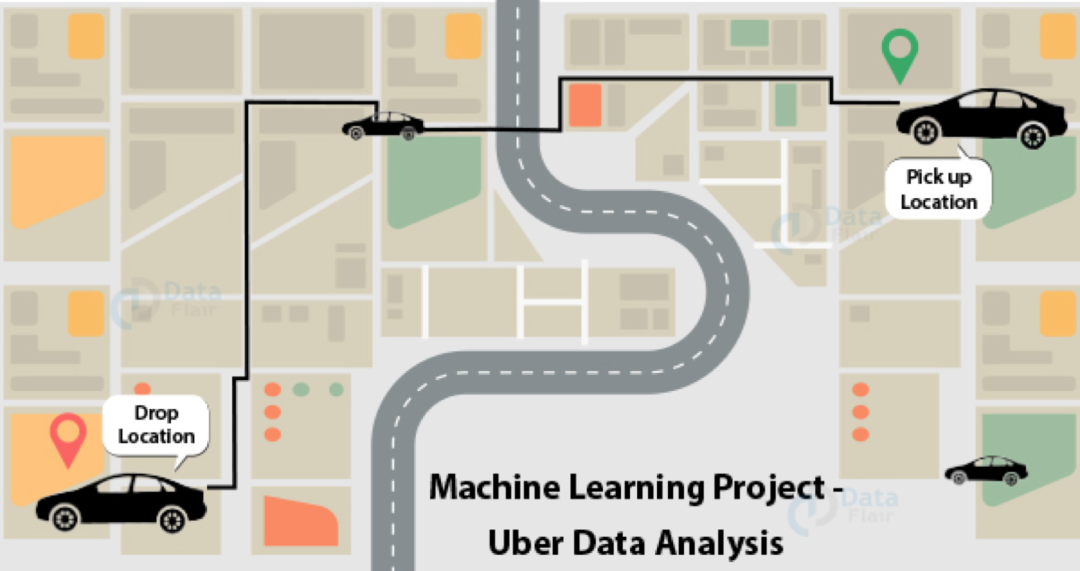项目构想：该项目可用于对超级数据执行数据可视化。该数据集包含纽约市中的450万个超级拾取器。为了分析行程，需要精美地表示很多数据，以便可以进一步改善业务。

数据集：Uber数据分析数据集

源代码：Uber数据分析项目

https://data-flair.training/blogs/r-data-science-project-uber-data-analysis/

### 4.人格预测项目

项目构想：Myers Briggs类型指示器是一种个性类型系统，根据内向，直觉，思维和感知能力将一个人分为16个不同的个性。我们可以根据他们在社交媒体上发布的帖子的类型来确定其个性。

数据集：个性预测数据集

https://www.kaggle.com/datasnaek/mbti-type

### 5. Xbox游戏预测项目

数据集：Xbox游戏预测数据集

https://www.kaggle.com/c/acm-sf-chapter-hackathon-small/overview

### 6.信用卡欺诈检测项目

项目构想：使用卡进行大量交易的公司需要发现系统中的异常情况。该项目旨在建立信用卡欺诈检测模型。我们将使用交易及其标签作为欺诈或非欺诈来检测客户进行的新交易是否为欺诈。

数据集：信用卡欺诈检测数据集

源代码：信用卡欺诈检测项目

https://data-flair.training/blogs/data-science-machine-learning-project-credit-card-fraud-detection/

### 7.芭比与大脑项目

项目构想：芭比娃娃等儿童玩具具有一组预定义的单词，可以重复说。我们可以使用机器学习方法使芭比娃娃有一些头脑。当玩具可以用不同的句子理解和说话时，它会更加吸引人。这是一个出色的项目，将改善孩子们的学习过程。

### 8.使用机器学习进行客户细分

项目构想：客户细分是一种根据客户的购买历史，性别，年龄，兴趣等对客户进行细分的技术。获取此信息非常有用，以便商店可以在个性化营销方面获得帮助，并为客户提供相关的信息交易。在该项目的帮助下，公司可以运行特定于用户的活动并提供特定于用户的报价，而不是向所有用户广播相同的报价。

数据集：客户细分数据集

源代码：客户细分项目

https://data-flair.training/blogs/r-data-science-project-customer-segmentation/

## 高级机器学习项目

### 1.使用机器学习进行情感分析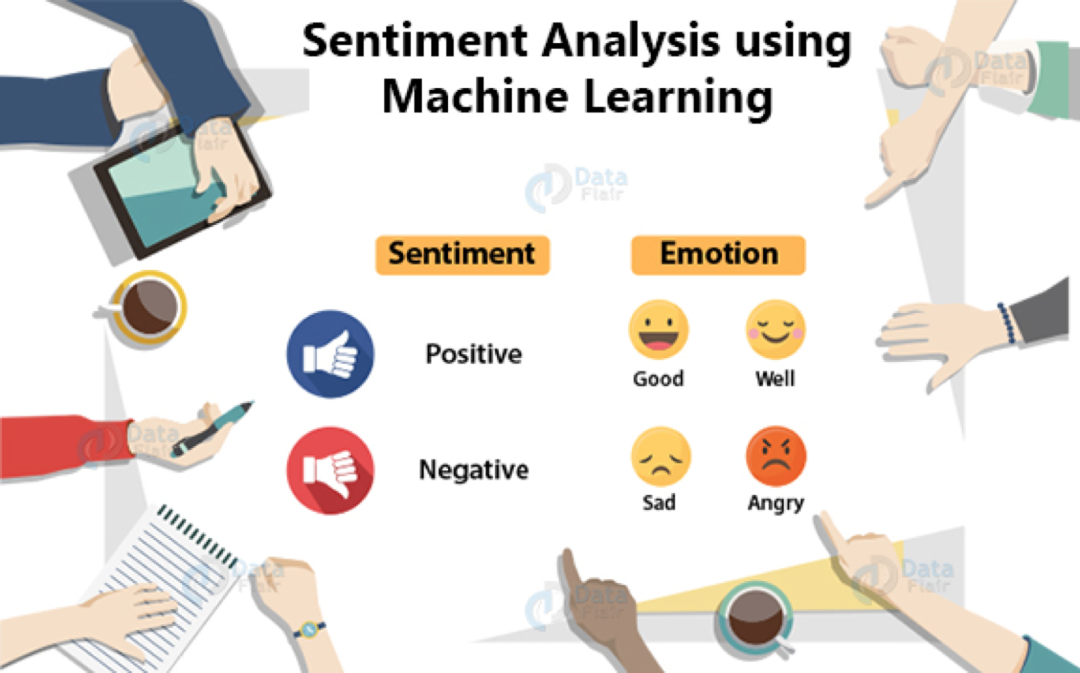项目构想：情感分析是分析用户情感的过程。我们可以将他们的情绪分为正面，负面或中性。了解如何进行情感分析是一个很棒的项目，并且如今已被广泛使用。这是最受欢迎的机器学习项目之一。其背后的原因是每家公司都试图了解客户的情绪，如果客户满意，他们会留下来。该项目可能显示出减少客户流失的途径。

数据集：情感分析数据集

http://ai.stanford.edu/~amaas/data/sentiment/

源代码：情感分析项目

https://data-flair.training/blogs/data-science-r-sentiment-analysis-project/

### 2.安然调查项目

项目构想：安然公司（Enron）在2000年倒闭，但可提供数据进行调查。该数据库包含500,000名在公司工作的真实员工的电子邮件，因此数据对于执行数据分析非常有用，许多数据科学家都使用此数据集。

数据集：安然调查数据集

https://www.cs.cmu.edu/~enron/

### 3.语音情感识别机器学习项目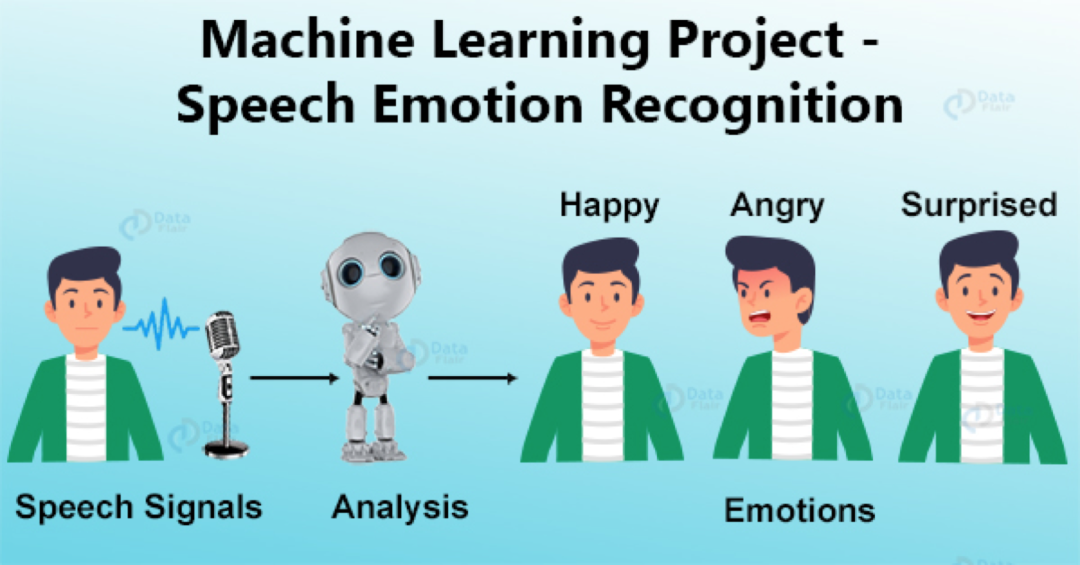项目构想：这是最好的机器学习项目之一。语音情感识别系统使用音频数据。它以语音的一部分作为输入，然后确定说话者以何种情绪说话。我们可以识别出不同的情绪，例如快乐，悲伤，惊讶，生气等。该项目可能有助于在与呼叫中心通话期间识别客户情绪。

数据集：语音情感识别数据集

源代码：语音情感识别项目

https://data-flair.training/blogs/python-mini-project-speech-emotion-recognition/

### 4.抓非法捕鱼项目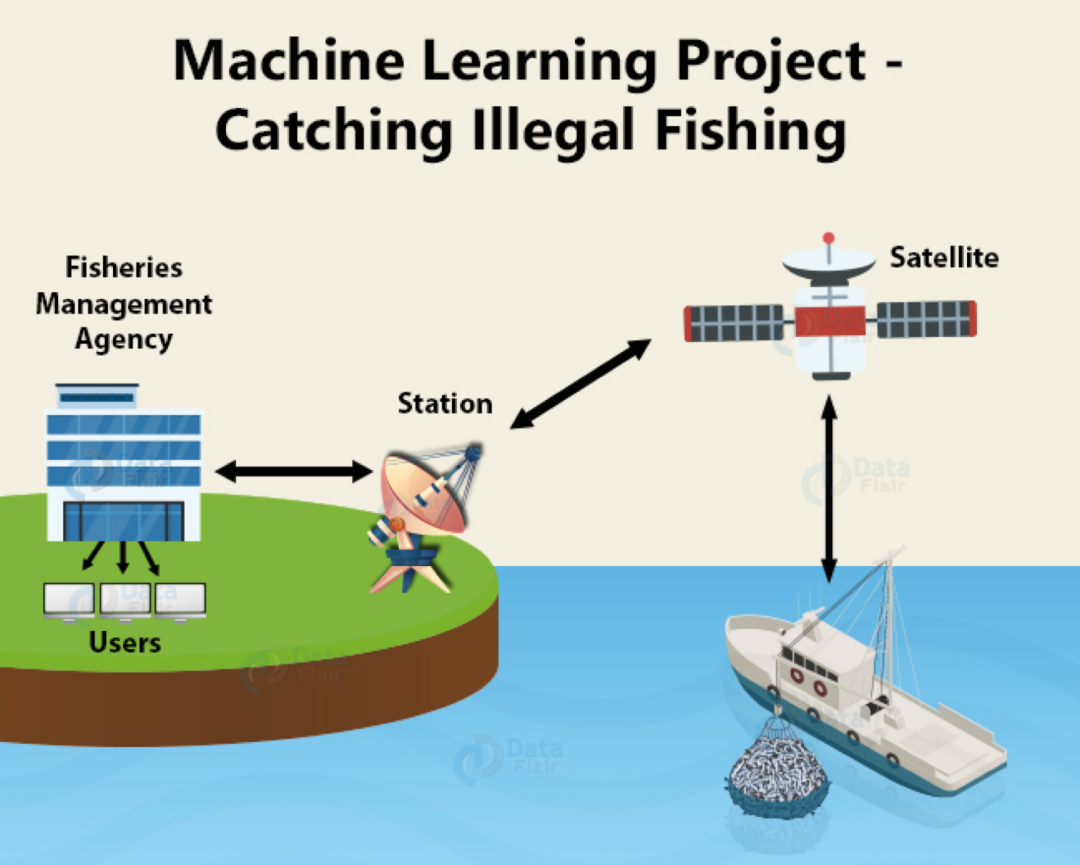项目构想：这是一个有趣的机器学习项目。海洋上有许多船，船，不可能手动跟踪每个人的活动。这将是一个了不起的项目，它可以通过卫星和地理位置数据识别非法偷猎动物并捕捞捕鱼活动。Global Fishing Watch免费提供实时数据，可用于构建系统。

数据集：捕捉非法捕鱼数据集

https://globalfishingwatch.org/map-and-data/

### 5.使用协同过滤的在线杂货推荐

项目构想：协作过滤是一项很棒的技术，可根据相似用户的反应来过滤出用户可能喜欢的项目。杂货店推荐系统将是一个使客户意识到自己想要的东西的好项目。

### 6.使用机器学习的电影推荐系统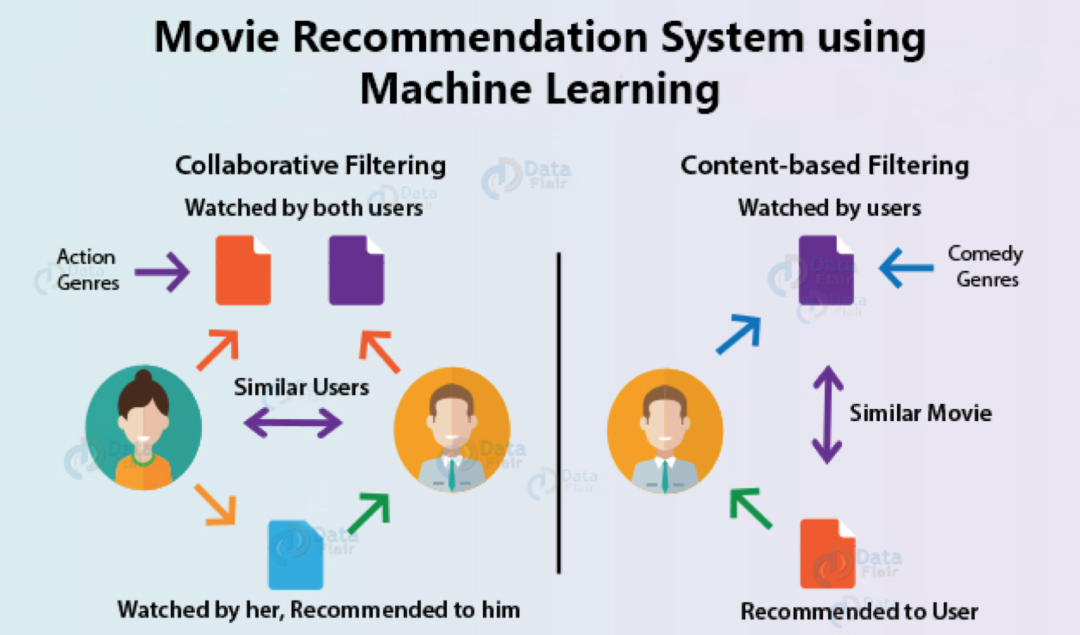项目构想：推荐系统无处不在，无论是在线购买应用程序，电影流媒体应用程序还是音乐流媒体。他们都根据目标客户推荐产品。电影推荐系统是增强你的作品集的绝佳项目。

数据集：电影推荐系统数据集

源代码：电影推荐系统项目

https://data-flair.training/blogs/data-science-r-movie-recommendation/

### 7.车牌自动识别系统

项目构想：该机器学习项目的目的是检测和识别车辆的牌照号，并读取印在牌照上的牌照号。这可能是用于安全扫描，流量监视等的良好应用程序。

源代码：自动车牌识别项目

展开全文深度学习
•   本篇主要是让大家熟悉机器学习实际项目中的全流程，并伴随案例讲解，通过让大家动手了解实际机器学习项目的大体流程，以及面对一些常见情况的处理方法 编程环境： Anaconda3：juputer notebook Python3 Pycharm...

### 前言

本篇主要是通过实例让大家熟悉机器学习实际项目中的全流程，通过让大家动手了解实际机器学习项目的大体流程，以及面对一些常见情况的处理方法。

本篇主要注重机器学习项目的全流程，所以其中的具体内容并没有展开，对于数据清洗、特征工程、算法原理可自行学习拓展。另外，文中难免会存在一些错误或代码报错（尤其是爬虫，可能会被禁止访问），这里我会提供用于本篇的代码和数据方便大家完整的了解机器学习全流程。

编程环境：

• Anaconda3：juputer notebook
• Python3
• Pycharm

本篇代码及数据：

#### 1、机器学习、深度学习与AI

（1）机器学习：

• 致力于通过研究如何通过 计算的手段，利用 经验 来改善系统自身的 性能，是人工智能的核心技术；

（2）深度学习

• 机器学习中 神经网络算法 的延伸，可以理解为包含 多个隐层 的神经网络模型

（3）人工智能（AI）

• 人工智能是利用 数字计算机 或者 数字计算机控制的机器 模拟、延伸和扩展人的智能，感知环境、获取知识并使用知识

#### 2、机器学习的核心任务

• 对于给定的 数据（结构化数据、文本、语音、视频或图像），通过设定一个 评价标准（损失函数），据此根据算法不断迭代训练进行学习，使得学习得到的 参数 能够使模型 最大程度拟合训练集同时具备最好的泛化能力，由这样的参数得到 最优的模型

#### 3、机器学习的核心要义

• 给定一组数据，要从数据中最大程度上归纳总结出普遍的规律
• 学习的不够，普遍规律没有归纳出来，这是欠拟合
• 学习能力太强，以至于将数据中的噪声也拟合了，这是过拟合
• 在模型空间中总存在一个模型，它能够最大程度的拟合训练数据，且对未知的测试数据具有最好的泛化能力
• 机器学习就是与欠拟合和过拟合做斗争
• 获取更多的数据、特征工程、正则化、算法调优便是有力的斗争武器

#### 4、机器学习项目全流程

• 需求分析：产品与技术调研
• 数据采集：自有数据/爬虫
• 数据清洗：占用大部分时间
• 数据分析与可视化：探索性数据分析、统计绘图
• 特征工程：建立在数据清洗基础上、特征选择与特征组合
• 机器学习建模与调优：各种模型各种调试
• 模型结果展示与报告：建模基础之上的决策

### 一、需求分析

#### 1、需求来源

• 项目需求：项目甲方、部门领导、项目产品经理
• 个人研究：个人

#### 2、需求分析

需求分析：结合【现有条件】将【需求方/业务方的问题】转化为一个【机器学习可解决的问题】

• 【现有条件】：包括企业或个人当前说具备的软硬件设备计算资源技术能力以及是否有数据（最重要的）
• 【需求方/业务方的问题】:要求需求承受者具备相应的软硬件计算和技术技能之外，还需要一定的专业领域和业务场景的知识
• 【机器学习可解决的问题】：通过对业务的分析和数据的分析找出关键特征，利用机器学习理论知识构建模型

可归纳为：数据、领域知识、机器学习技术能力

#### 3、产品和技术调研

• 数据科学竞赛层面：有无项目类似的公开竞赛
• 论文层面：国内外（主要是国外）有无项目相关领域和方向的研究论文
• 产品应用层面：国内外是否有项目相关的产品和应用范例

#### 4、实例：数据科学相关岗位的薪资水平

哪些岗位属于数据科学相关岗位，可以列出多个关键字：数据分析师、数据挖掘工程师、大数据工程师、机器学习算法工程师、深度学习算法工程师等### 二、数据采集

【数据获取方法：】

• 企业自有数据源进行采集
• 抓取采集网络数据（爬虫）
• 直接从数据库提取数据
• 从统计年报等获取数据
• 开源数据集：uci机器学习库/kaggle datasets

#### 1、实例：爬取拉勾网数据

拉勾网有一个比较奇怪的地方，从导航页点击岗位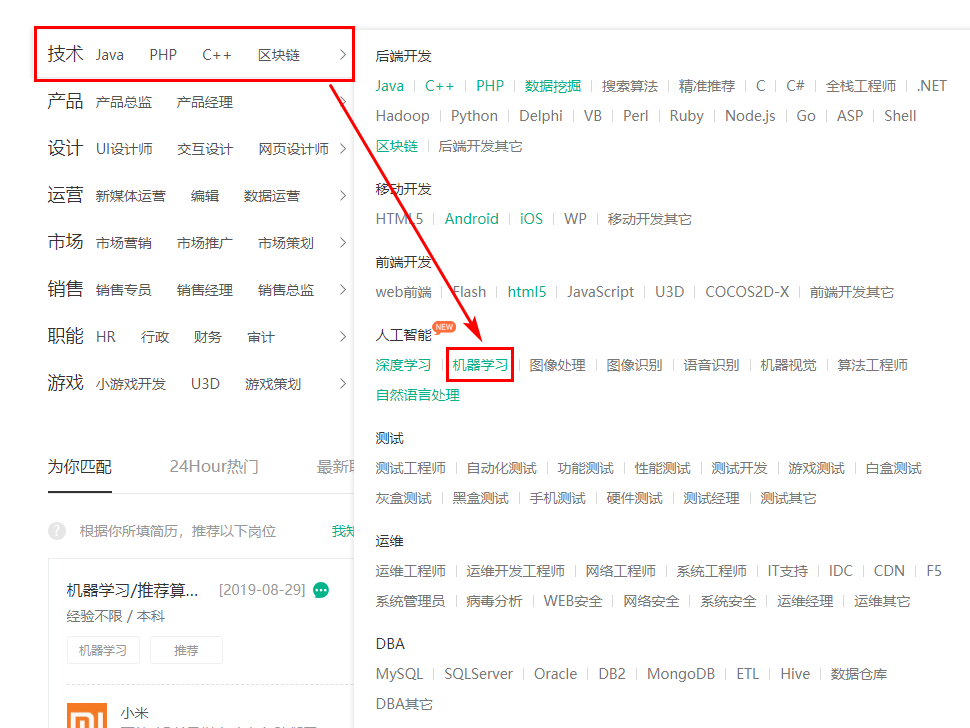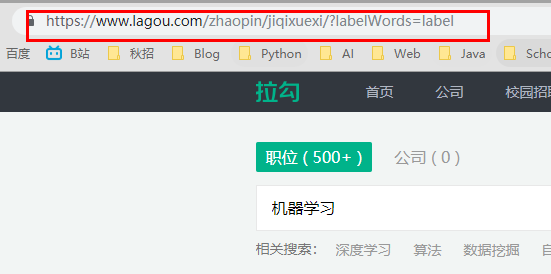尝试切换下一页观察网址的变化，发现网址是变化的，这表示页面是静态的

如果通过搜索框搜索：机器学习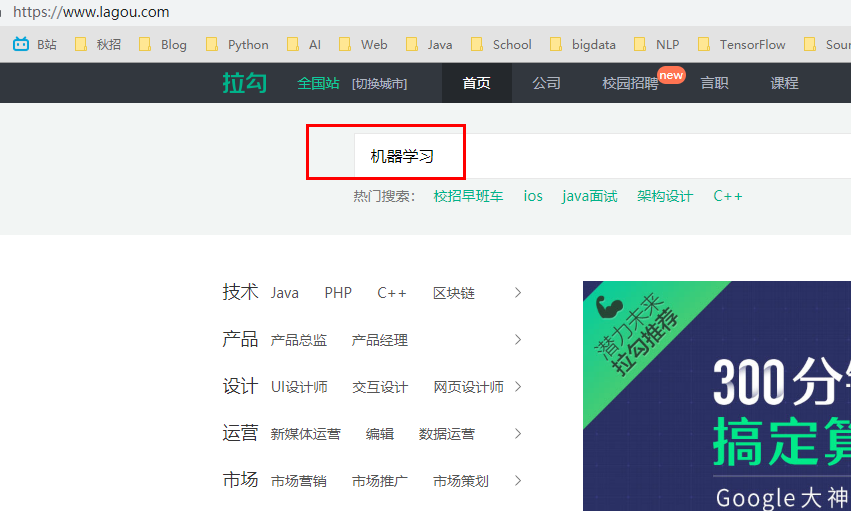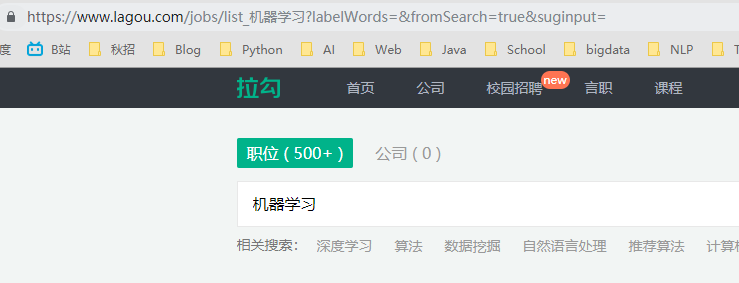同样切换页数，发现网址没发生变化，说明页面是动态的

下面我们分别针对两种页面进行爬虫：

静态数据采集：

（1）导入相关库以及参数设置

# 导入相关库
import requests
from lxml import etree
import pandas as pd
from time import sleep
import random

'User-Agent': 'Mozilla/5.0 (Windows NT 10.0; Win64; x64) AppleWebKit/537.36 (KHTML, like Gecko) Chrome/68.0.3440.106 Safari/537.36',
}（2）看网页结构循环页数进行采集（以机器学习关键字为例）

1. url：
• 第一页：https://www.lagou.com/zhaopin/jiqixuexi/1/?filterOption=3
• 第二页：https://www.lagou.com/zhaopin/jiqixuexi/2/?filterOption=3
• 归纳：url = 'https://www.lagou.com/zhaopin/jiqixuexi/{}/?filterOption=3'.format(i)
1. job_name其他也类似，这里就不一一举出

直接上代码：

for i in range(1, 6):
sleep(random.randint(3, 10))
url = 'https://www.lagou.com/zhaopin/jiqixuexi/{}/?filterOption=3'.format(i)
print('正在抓取第{}页...'.format(i), url)
# 请求网页并解析
# 使用xpath表达式抽取各目标字段
job_name = [i for i in con.xpath("//a[@class='position_link']/h3/text()")]
job_company = [i for i in con.xpath("//div[@class='company_name']/a/text()")]
job_salary = [i for i in con.xpath("//span[@class='money']/text()")]
job_exp_edu = [i for i in con.xpath("//div[@class='li_b_l']/text()")]
job_exp_edu2 = [i for i in [i.strip() for i in job_exp_edu] if i != '']
job_industry = [i for i in con.xpath("//div[@class='industry']/text()")]
job_tempation = [i for i in con.xpath("//div[@class='list_item_bot']/div[@class='li_b_r']/text()")]
job_links = [i for i in con.xpath("//div[@class='p_top']/a/@href")]

# 获取详情页链接后采集详情页岗位描述信息
job_des = []
sleep(random.randint(3, 10))
des = [[i.xpath('string(.)') for i in con2.xpath("//dd[@class='job_bt']/div/p")]]
job_des += des

break

# 对数据进行字典封装
dataset = {
'岗位名称': job_name,
'公司': job_company,
'薪资': job_salary,
'经验学历': job_exp_edu2,
'所属行业': job_industry,
'岗位福利': job_tempation,
'任职要求': job_des
}

# 转化为数据框并存为csv
data = pd.DataFrame(dataset)
data.to_csv('machine_learning_hz_job2.csv')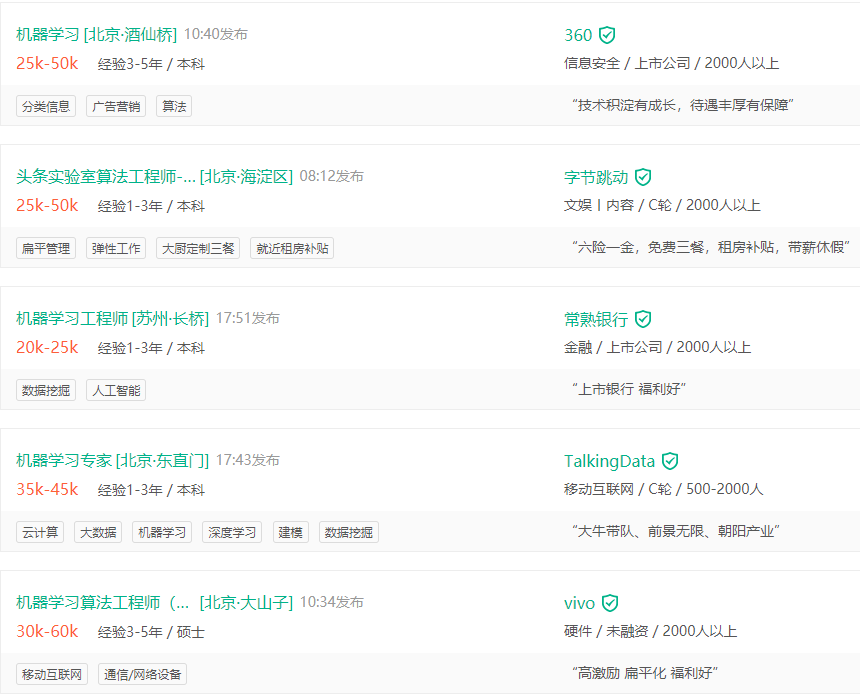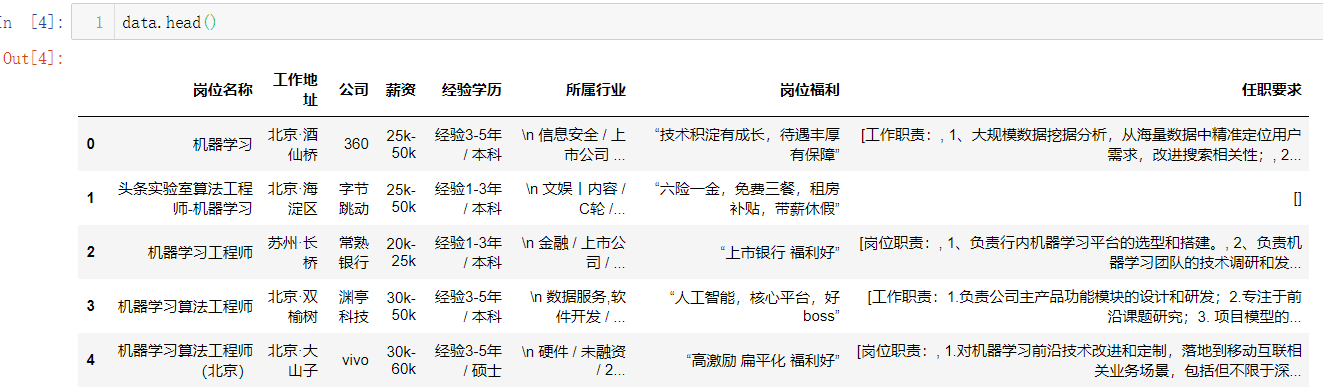上图可见，数据是一一对应的，表示我们爬取到了数据

函数封装：

# 函数化封装
import requests
from lxml import etree
import pandas as pd
from time import sleep
import random

def static_crawl():
'User-Agent': 'Mozilla/5.0 (Windows NT 10.0; Win64; x64) AppleWebKit/537.36 (KHTML, like Gecko) Chrome/68.0.3440.106 Safari/537.36',
}

for i in range(1, 7):
sleep(random.randint(3, 10))
url = 'https://www.lagou.com/zhaopin/jiqixuexi/{}/?filterOption=3'.format(i)
print('正在抓取第{}页...'.format(i), url)

job_name = [i for i in con.xpath("//a[@class='position_link']/h3/text()")]
job_company = [i for i in con.xpath("//div[@class='company_name']/a/text()")]
job_salary = [i for i in con.xpath("//span[@class='money']/text()")]
job_exp_edu = [i for i in con.xpath("//div[@class='li_b_l']/text()")]
job_exp_edu2 = [i for i in [i.strip() for i in job_exp_edu] if i != '']
job_industry = [i for i in con.xpath("//div[@class='industry']/text()")]
job_tempation = [i for i in con.xpath("//div[@class='list_item_bot']/div[@class='li_b_r']/text()")]
job_links = [i for i in con.xpath("//div[@class='p_top']/a/@href")]

job_des = []
sleep(random.randint(3, 10))
des = [[i.xpath('string(.)') for i in con2.xpath("//dd[@class='job_bt']/div/p")]]
job_des += des

lagou_dict = {
'岗位名称': job_name,
'公司': job_company,
'薪资': job_salary,
'经验学历': job_exp_edu2,
'所属行业': job_industry,
'岗位福利': job_tempation,
'任职要求': job_des
}

crawl_data = pd.DataFrame(lagou_dict)
data.to_csv('machine_learning_hz_job2.csv')

return crawl_data


动态数据采集：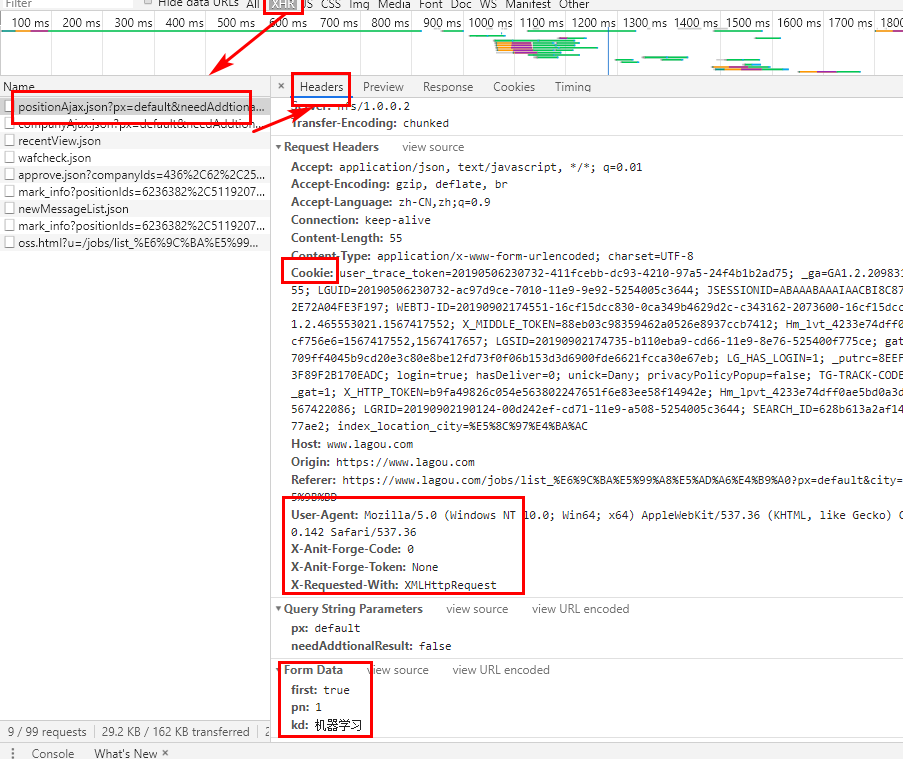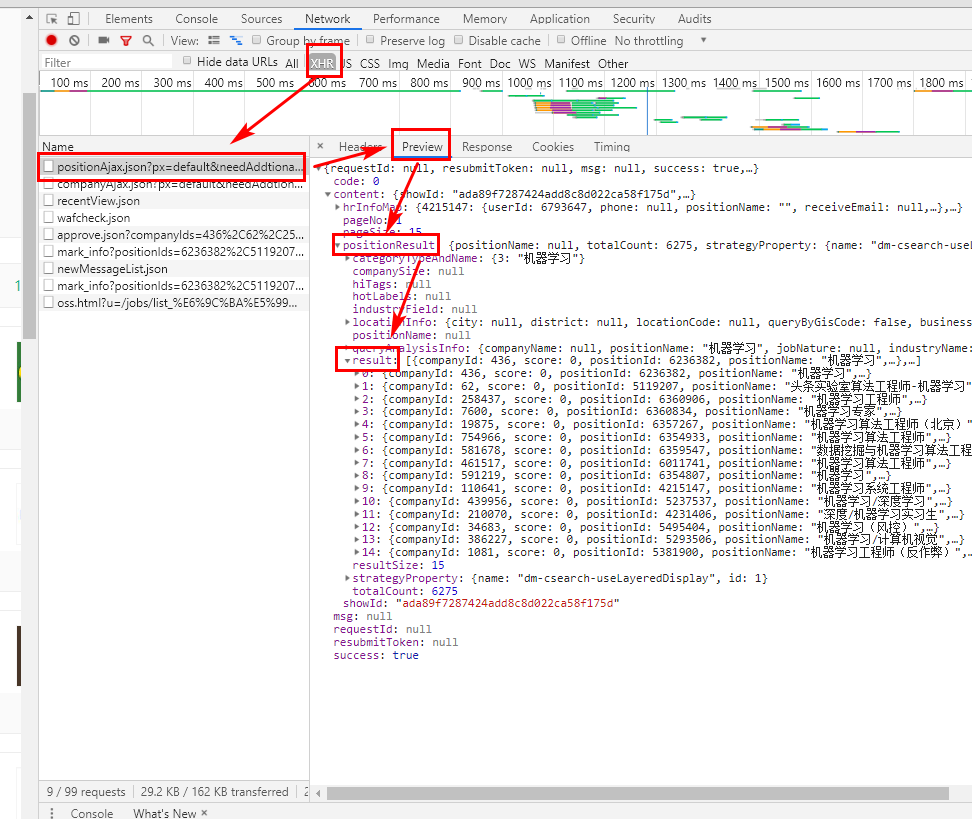import json
import time
import requests
from bs4 import BeautifulSoup
import pandas as pd

#定义抓取主函数
def lagou_dynamic_crawl():
'User-Agent':'Mozilla/5.0 (Windows NT 10.0; Win64; x64) AppleWebKit/537.36 (KHTML, like Gecko) Chrome/75.0.3770.142 Safari/537.36',
'Host':'www.lagou.com',
'X-Anit-Forge-Code':'0',
'X-Anit-Forge-Token':None,
'X-Requested-With':'XMLHttpRequest',
}

#创建一个职位列表容器
positions = []
#30页循环遍历抓取
for page in range(1, 31):
print('正在抓取第{}页...'.format(page))
#构建请求表单参数
params = {
'first':'true',
'pn':page,
'kd':'机器学习'
}

#构造请求并返回结果
#将请求结果转为json
json_result = result.json()
print(json_result)
#解析json数据结构获取目标信息
position_info = json_result['content']['positionResult']['result']
#循环当前页每一个职位信息，再去爬职位详情页面
for position in position_info:
#把我们要爬取信息放入字典
position_dict = {
'position_name':position['positionName'],
'work_year':position['workYear'],
'education':position['education'],
'salary':position['salary'],
'city':position['city'],
'company_name':position['companyFullName'],
'label':position['companyLabelList'],
'stage':position['financeStage'],
'size':position['companySize'],
'industry':position['industryField'],
'industryLables':position['industryLables']
}
#找到职位 ID
position_id = position['positionId']
#根据职位ID调用岗位描述函数获取职位JD
position_dict['position_detail'] = recruit_detail(position_id)
positions.append(position_dict)

time.sleep(4)
print('全部数据采集完毕。')
return positions

#定义抓取岗位描述函数
def recruit_detail(position_id):
'User-Agent':'Mozilla/5.0 (Windows NT 10.0; Win64; x64) AppleWebKit/537.36 (KHTML, like Gecko) Chrome/75.0.3770.142 Safari/537.36',
'Host':'www.lagou.com',
}
url = 'https://www.lagou.com/jobs/%s.html' % position_id
time.sleep(5)
#解析职位要求text
soup = BeautifulSoup(result.text, 'html.parser')
job_jd = soup.find(class_="job_bt")

#通过尝试发现部分记录描述存在空的情况
#所以这里需要判断处理一下
if job_jd != None:
job_jd = job_jd.text
else:
job_jd = 'null'
return job_jd

if __name__ == '__main__':
positions = lagou_dynamic_crawl()
df = pd.DataFrame(positions)
df.to_csv('data_mining_hz.csv')


### 三、数据清洗（脏数据）

脏数据可以理解为带有不整洁程度的原始数据；原始数据的整洁程度由数据采集质量所决定。脏数据的表现形式五花八门，如若数据采集质量不过关，拿到的原始数据内容只有更差没有最差。

【脏数据的表现形式包括：】

• 数据串行，尤其是长文本情形下
• 数值变量种混有文本/格式混乱
• 各种符号乱入
• 数据记录错误
• 大段缺失（某种意义上不算脏数据）

数据采集完后拿到的原始数据到建模前的数据是一个十分耗时的过程，从数据分析的角度上来讲，这个中间处理脏数据的数据预处理和清洗过程几乎占到了我们全部机器学习项目的60%-70%的时间。

总体而言就是 原始数据 -> 基础数据预处理/清洗 -> 探索性数据分析 -> 统计绘图/数据可视化 -> 特征工程

#### 1、数据清洗与预处理基本方向

• 数据预处理没有特别固定的套路
• 数据预处理的困难程度与原始数据脏的程度而定
• 原始数据越脏，数据预处理工作越艰辛
• 数据预处理大的套路没有，小的套路一大堆
• 机器学习的数据预处理基于pandas来做
• 缺失值处理
• 小文本和字符串数据处理
• 法无定法，融会贯通

缺失值处理方法：

• 删除:超过70%以上的缺失
• 填充

小文本与字符串处理：

• python 字符串处理函数
• 正则表达式

#### 2、招聘数据的清洗过程

import numpy as np
import pandas as pd
import warnings
warnings.filterwarnings('ignore')

data = pd.concat((pd.concat((pd.concat((data1, data2)), data3)), data4)).reset_index(drop=True)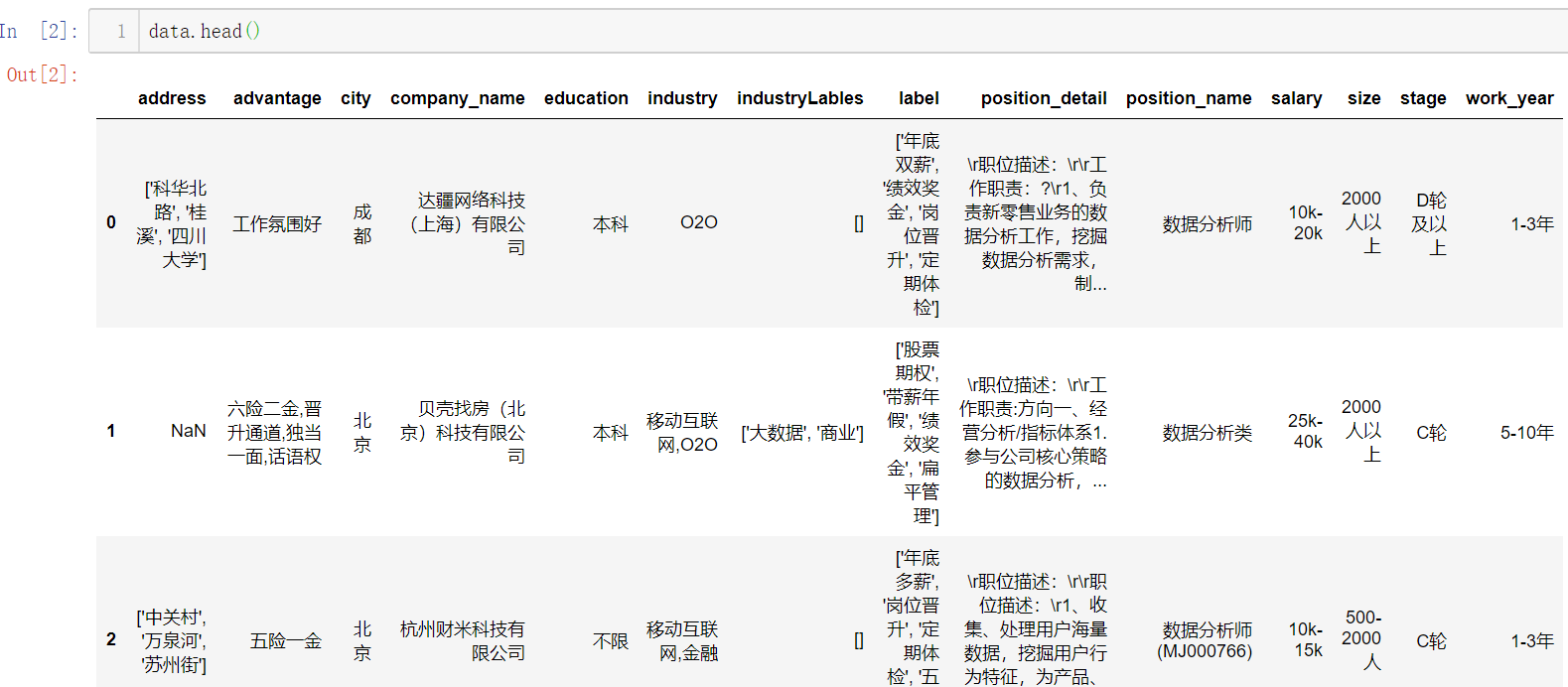【展示数据信息，可以看出哪些特征有缺失值：】【给位置地址的填充['未知']【去掉[]：】【将回车符 \r替换为空：】【填充未知，并替换回车符：】至此可以发现，已经没有缺省值，并且去掉了 []

【工资特征处理：】

for i, j in enumerate(data['salary']):
j = j.replace('k', '').replace('K', '').replace('以上', '-0')
j1 = int(j.split('-'))
j2 = int(j.split('-'))
j3 = 1/2 * (j1+j2)
data['salary'][i] = j3*1000



将原本字符串格式，转为数值型，取工资范围的平均值

【公司规模、公司发展、工作年限这三个特征取值不多，可以不做处理，后面直接进行哑编码/One-hot编码：】【岗位名称特征处理：】

for i, j in enumerate(data['position_name']):
if '数据分析' in j:
j = '数据分析师'
if '数据挖掘' in j:
j = '数据挖掘工程师'
if '机器学习' in j:
j = '机器学习工程师'
if '深度学习' in j:
j = '深度学习工程师'
data['position_name'][i] = j
data['position_name'][:5]


代码模块化：

import numpy as np
import pandas as pd
import string
import warnings
warnings.filterwarnings('ignore')

class data_clean(object):
def __init__(self):
pass

def get_data(self):

data = pd.concat((pd.concat((pd.concat((data1, data2)), data3)), data4)).reset_index(drop=True)
return data

def clean_operation(self):
data = self.get_data()
j = j.replace('[', '').replace(']', '')

for i, j in enumerate(data['salary']):
j = j.replace('k', '').replace('K', '').replace('以上', '-0')
j1 = int(j.split('-'))
j2 = int(j.split('-'))
j3 = 1/2 * (j1+j2)
data['salary'][i] = j3*1000

for i, j in enumerate(data['industryLables']):
j = j.replace('[', '').replace(']', '')
data['industryLables'][i] = j

for i, j in enumerate(data['label']):
j = j.replace('[', '').replace(']', '')
data['label'][i] = j

data['position_detail'] = data['position_detail'].fillna('未知')
for i, j in enumerate(data['position_detail']):
j = j.replace('\r', '')
data['position_detail'][i] = j

return data

opt = data_clean()
data = opt.clean_operation()


### 四、数据分析与可视化

#### 1、探索性数据分析（Explore data analysis）

EDA一定程度上跟描述性数据分析重合，但范围要大于描述性数据分析。不清楚数据长什么样、不知道数据里有什么，目标不甚明确，大目标清晰，中间途径不清晰，需要从数据中找到线索。

EDA是一种方法论而不是特定技术

【探索性数据分析的基本面：】

• 定量分析展示
• 统计绘图与数据可视化

【探索性数据分析的具体方法：】

• 数据基本概况（统计定量分析）
• 缺失值展示与探索
• 异常值和离群点展示与探索
• 目标变量重点分析
• 自变量逐变量分析（数值型、分类型、文本特征）
• 自变量目标变量相关分析
• 统计绘图与可视化展示

#### 2、统计绘图与数据可视化

【现代统计图形（最常用）：】

• 柱状图/条形图：单变量/多变量
• 散点图：单变量/多变量
• 直方图：单变量，尤其是数值型目标变量的分布展示
• 箱线图：离散变量与连续变量的关系展示
• 折线图：单变量/多变量
• 密度图：和直方图联用

【数据可视化技术：】

• 统计图形
• R语言可视化：ggplot2
• python可视化：matplotlibseabornbokehplotlywordcloud
• web可视化：交互式绘图echartsjavascriptD3.js
• 地图可视化

对于数据分析和机器学习而言，掌握前三个即可，重点在于各种统计图形。

#### 3、实例：招聘数据的探索性数据分析

import pandas as pd
import numpy as np
import matplotlib.pyplot as plt
import seaborn as sns



【数据概况：】【目标变量分析：】# 计算目标变量值的偏度与峰度
from scipy import stats
# from scipy.stats import norm

print("Skewness: %f" % data['salary'].skew())
print("Kurtosis: %f" % data['salary'].kurt())


【分类值统计：】

cols = ['city', 'education', 'position_name', 'size', 'stage', 'work_year']
for col in cols:
print(data[col].value_counts())

# 处理city变量
# 将计数少于30的划为其他
city_counts_df = pd.DataFrame()
city_counts_df['city'] = city_counts.index
city_counts_df['counts'] = data['city'].value_counts().values
cities = ['北京', '上海', '广州', '深圳', '杭州', '成都', '武汉', '南京']
for i, j in enumerate(data['city']):
if j not in cities:
data['city'][i] = '其他'北京薪资水平明显高一个档次博士工资水平明显高工作年限越长，工资水平越高图中，上市公司与D轮及以上稍微高点，B轮的也不低，其实说明有些时候公司在发展中时如果资金允许更愿意多花点钱招入人才其中，2000人以上的比较高，少于15人的也比较高，可能因为人少的公司一个人要当几个人用，所以工资会给的高点机器学习、数据挖掘、深度学习差不多

# 处理industry变量
for i, j in enumerate(data['industry']):
if ',' not in j:
data['industry'][i] = j
else:
data['industry'][i] = j.split(',')

#data['industry'].value_counts()

industries = ['移动互联网', '金融', '数据服务', '电子商务', '企业服务', '医疗健康', 'O2O', '硬件', '信息安全', '教育']
for i, j in enumerate(data['industry']):
if j not in industries:
data['industry'][i] = '其他'

#data['industry'].value_counts()O2O、医疗健康的工资水平较高

【岗位待遇特征处理：】

• 因为这是个长文本，这里采用了分词，取关键词，画词云图
ADV = []

import jieba
print("切分结果:  "+",".join(result))

jieba.suggest_freq(('五险一金'), True)
jieba.suggest_freq(('六险一金'), True)
jieba.suggest_freq(('带薪年假'), True)
jieba.suggest_freq(('年度旅游'), True)
jieba.suggest_freq(('氛围好'), True)
jieba.suggest_freq(('技术大牛'), True)
jieba.suggest_freq(('免费三餐'), True)
jieba.suggest_freq(('租房补贴'), True)
jieba.suggest_freq(('大数据'), True)
jieba.suggest_freq(('精英团队'), True)
jieba.suggest_freq(('晋升空间大'), True)

print("切分结果:  "+",".join(result))

#读取标点符号库
f = open("./stopwords.txt", "r")
f.close()
#加载用户自定义词典
mytext_list=[]
#文本清洗
for seg in segs:
if seg not in stopwords and seg != " " and len(seg) != 1:
mytext_list.append(seg.replace(" ", ""))

from wordcloud import WordCloud
wc = WordCloud(
background_color="white", #背景颜色
max_words=800, #显示最大词数
font_path = r'C:\Windows\Fonts\STFANGSO.ttf',
min_font_size=15,
max_font_size=80
)
plt.imshow(wc)
plt.show()# 剔除几个无用变量
data2 = data.drop(['address', 'industryLables', 'company_name'], axis=1)
data2.shape  # (1650, 11)


### 五、特征工程

#### 1、特征工程概述

什么是特征工程？特征工程指的是最大程度上从原始数据中汲取特征和信息来使得模型和算法达到尽可能好的效果。

【特征工程具体内容包括：】

• 数据预处理
• 特征选择
• 特征变换与提取
• 特征组合
• 数据降维

【特征工程的两个基本面：】

• 基于数理和模型的考虑
• 基于业务的考虑（需要了解数据所属业务领域的专业知识）

【几个重要观点：】

• 在实际的特征工程实践中，这两个基本面都要考虑，尤其是业务层面，直接关乎到模型的表现。
• 在数据维度特别大、特征数量极多的情况下，找到数据中的 magic feature 至关重要。
• 总之，数据和特征决定了机器学习效果的上限，模型和算法只是不断地逼近这个上限而已。
• kaggle、天池等数据科学竞赛比模型吗？比算法吗？通通不是，比的是特征工程。

#### 2、数据预处理

• 一些前期的数据清洗和预处理工作，是对原始数据的基本整理和重塑

#### 3、特征选择

特征选择即选择与目标变量相关的自变量进行用于建模，也叫变量筛选。

【特征选择基于两个基本面：】

• 特征是否发散，即该特征对于模型是否有解释力，如果特征是一成不变的（0方差），这样的特征是无用的。
• 特征是否与目标变量有一定的相关性。这一点要充分基于业务层面去考虑。

【特征选择柳叶三刀：】

• Filter (飞刀)：特征过滤
• Wrapper (弯刀)：特征包装
• Embedded (电刀)：特征嵌入

除了基于pandas和numpy的手动特征选择外，sklearn也有一套特征选择模块。

# 过滤法之方差筛选
from sklearn.feature_selection import VarianceThreshold
X = [[0, 0, 1], [0, 1, 0], [1, 0, 0], [0, 1, 1], [0, 1, 0], [0, 1, 1]]
sel = VarianceThreshold(threshold=(.8 * (1 - .8)))
sel.fit_transform(X)


第一列值为0的比例超过了80%，在结果中VarianceThreshold剔除这一列

# 过滤法之卡方检验
from sklearn.feature_selection import SelectKBest
from sklearn.feature_selection import chi2
X, y = iris.data, iris.target
X.shape
X_new = SelectKBest(chi2, k=2).fit_transform(X, y)
X_new.shape


通过卡方检验筛选2个最好的特征

# 包装法
# 选定一些算法，根据算法在数据上的表现来选择特征集合，一般选用的算法包括随机森林、支持向量机和k近邻等常用算法。
import matplotlib.pyplot as plt
from sklearn.svm import SVC
from sklearn.model_selection import StratifiedKFold
from sklearn.feature_selection import RFECV
from sklearn.datasets import make_classification

X, y = make_classification(n_samples=1000, n_features=25, n_informative=3,n_redundant=2,
n_repeated=0, n_classes=8, n_clusters_per_class=1, random_state=0)

svc = SVC(kernel="linear")
rfecv = RFECV(estimator=svc, step=1, cv=StratifiedKFold(2), scoring='accuracy')
rfecv.fit(X, y)

print("Optimal number of features : %d" % rfecv.n_features_)

plt.figure()
plt.xlabel("Number of features selected")
plt.ylabel("Cross validation score (nb of correct classifications)")
plt.plot(range(1, len(rfecv.grid_scores_) + 1), rfecv.grid_scores_)
plt.show();可以发现特征数量在3的时候达到最高

# 嵌入法之基于惩罚项的特征选择法
from sklearn.svm import LinearSVC
from sklearn.feature_selection import SelectFromModel
X, y = iris.data, iris.target
print('原始数据特征维度：', X.shape)
lsvc = LinearSVC(C=0.01, penalty="l1", dual=False).fit(X, y)
model = SelectFromModel(lsvc, prefit=True)
X_new = model.transform(X)
print('l1惩罚处理之后的数据维度：', X_new.shape)


原始数据特征维度： (150, 4)
l1惩罚处理之后的数据维度： (150, 3)

# 嵌入法之基于树模型的特征选择法
from sklearn.ensemble import ExtraTreesClassifier
from sklearn.feature_selection import SelectFromModel
X, y = iris.data, iris.target
print('原始数据特征维度：', X.shape)
clf = ExtraTreesClassifier()
clf = clf.fit(X, y)
clf.feature_importances_
model = SelectFromModel(clf, prefit=True)
X_new = model.transform(X)
print('l1惩罚处理之后的数据维度：', X_new.shape)


原始数据特征维度： (150, 4)
l1惩罚处理之后的数据维度： (150, 2)

#### 4、特征变换与特征提取

【数据特征逐个处理：】

• 数据标准化：基于列
• 数据区间缩放
• 数据归一化：基于行
• 数值目标变量对数化处理（有必要的情况下）
• 定量特征二值化（有必要的情况下）
• 定性特征哑编码（one-hot）
• 大文本信息提取（效果类似于one-hot）
# one-hot的两种方法
# sklearn onehotencoder
from sklearn.preprocessing import OneHotEncoder
OneHotEncoder().fit_transform(iris.target.reshape((-1,1))).toarray()

# pandas dummies 方法
import pandas as pd
pd.get_dummies(iris.target)


#### 5、特征组合

在单特征不能取得进一步效果的情况下可尝试不同特征之间的特征组合。特别需要基于业务考量，而不是随意组合。

#### 6、降维

适用于高维数据，成千上万的特征数量，但一般特征情况下不建议使用。

• PCA
• SVD
• LDA
• t-SNE

#### 7、实例：招聘数据的特征工程探索

import warnings
warnings.filterwarnings('ignore')
import numpy as np
import pandas as pd

# 分类变量one-hot处理
# pandas one-hot方法

# sklearn onehot方法
# 先要硬编码labelcoder
from sklearn.preprocessing import OneHotEncoder
from sklearn.preprocessing import LabelEncoder
lbl = LabelEncoder()
lbl.fit(list(lagou_df['city'].values))
lagou_df['city'] = lbl.transform(list(lagou_df['city'].values))
# 查看硬编码结果
# 再由硬编码转为one-hot编码
df_city = OneHotEncoder().fit_transform(lagou_df['city'].values.reshape((-1,1))).toarray()
#df_city[:5]

# 分类特征统一one-hot处理
cat_features = ['city', 'industry', 'education', 'position_name', 'size', 'stage', 'work_year']
for col in cat_features:
temp = pd.get_dummies(lagou_df[col])
lagou_df = pd.concat([lagou_df, temp],axis=1)
lagou_df = lagou_df.drop([col], axis=1)

#lagou_df.shape

pd.options.display.max_columns = 999


#### 8、职位描述特征的信息提取

lagou_df2 = pd.read_csv('./lagou_data5.csv', encoding='gbk')
lagou_df2 = lagou_df2[['position_detail', 'salary']]

# 提取Python信息
for i, j in enumerate(lagou_df2['position_detail']):
if 'python' in j:
lagou_df2['position_detail'][i] = j.replace('python', 'Python')

lagou_df2['Python'] = pd.Series()
for i, j in enumerate(lagou_df2['position_detail']):
if 'Python' in j:
lagou_df2['Python'][i] = 1
else:
lagou_df2['Python'][i] = 0

lagou_df2['Python'][:20]

# 提取R信息
lagou_df2['R'] = pd.Series()
for i, j in enumerate(lagou_df2['position_detail']):
if 'R' in j:
lagou_df2['R'][i] = 1
else:
lagou_df2['R'][i] = 0

lagou_df2['R'].value_counts()

# 提取SQL信息
for i, j in enumerate(lagou_df2['position_detail']):
if 'sql' in j:
lagou_df2['position_detail'][i] = j.replace('sql', 'SQL')

lagou_df2['SQL'] = pd.Series()
for i, j in enumerate(lagou_df2['position_detail']):
if 'SQL' in j:
lagou_df2['SQL'][i] = 1
else:
lagou_df2['SQL'][i] = 0

lagou_df2['SQL'].value_counts()

# 提取Excel信息
lagou_df2['Excel'] = pd.Series()
for i, j in enumerate(lagou_df2['position_detail']):
if 'Excel' in j:
lagou_df2['Excel'][i] = 1
else:
lagou_df2['Excel'][i] = 0

lagou_df2['Excel'].value_counts()

# 提取Java信息
lagou_df2['Java'] = pd.Series()
for i, j in enumerate(lagou_df2['position_detail']):
if 'Java' in j:
lagou_df2['Java'][i] = 1
else:
lagou_df2['Java'][i] = 0

lagou_df2['Java'].value_counts()

# 提取Linux信息
for i, j in enumerate(lagou_df2['position_detail']):
if 'linux' in j:
lagou_df2['position_detail'][i] = j.replace('linux', 'Linux')

lagou_df2['Linux'] = pd.Series()
for i, j in enumerate(lagou_df2['position_detail']):
if 'Linux' in j:
lagou_df2['Linux'][i] = 1
else:
lagou_df2['Linux'][i] = 0

lagou_df2['Linux'].value_counts()

# 提取C++信息
lagou_df2['C++'] = pd.Series()
for i, j in enumerate(lagou_df2['position_detail']):
if 'C++' in j:
lagou_df2['C++'][i] = 1
else:
lagou_df2['C++'][i] = 0

lagou_df2['C++'].value_counts()

# 提取Spark信息
for i, j in enumerate(lagou_df2['position_detail']):
if 'spark' in j:
lagou_df2['position_detail'][i] = j.replace('spark', 'Spark')

lagou_df2['Spark'] = pd.Series()
for i, j in enumerate(lagou_df2['position_detail']):
if 'Spark' in j:
lagou_df2['Spark'][i] = 1
else:
lagou_df2['Spark'][i] = 0

lagou_df2['Spark'].value_counts()

# 提取Tensorflow信息
for i, j in enumerate(lagou_df2['position_detail']):
if 'tensorflow' in j:
lagou_df2['position_detail'][i] = j.replace('tensorflow', 'Tensorflow')

if 'TensorFlow' in j:
lagou_df2['position_detail'][i] = j.replace('TensorFlow', 'Tensorflow')

lagou_df2['Tensorflow'] = pd.Series()
for i, j in enumerate(lagou_df2['position_detail']):
if 'Tensorflow' in j:
lagou_df2['Tensorflow'][i] = 1
else:
lagou_df2['Tensorflow'][i] = 0

lagou_df2['Tensorflow'].value_counts()

lagou_df2 = lagou_df2.drop(['position_detail'], axis=1)lagou_df = lagou_df.drop(['position_detail', 'salary'], axis=1)

lagou = pd.concat((lagou_df2, lagou_df), axis=1).reset_index(drop=True)
lagou.to_csv('lagou_featured.csv', encoding='gbk')


### 六、机器学习建模与调优

【模型与算法】

• 模型：一类问题的解题步骤，即一类问题的算法。
• 算法：能够解决特定问题的无歧义、机械、有效的运算流程和规则。

【机器学习中的三要素】

• 模型、策略与算法
• 模型：回归模型、分类模型
• 算法：有了模型和策略之后的优化算法：梯度下降法、牛顿法

【机器学习模型】

• 传统机器学习模型（单模型）
• 集成(ensemble)与提升（boosting）模型
• 神经网络与深度学习• 预处理
• 降维
• 分类
• 回归
• 聚类
• 模型评估与选择

#### 2、机器学习调参

【机器学习模型的参数有哪些？】

（1）模型训练参数：机器学习需要学习的东西，由训练得出，无需也无法调整

• 神经网络的权重与偏置
• 线性回归的变量系数

（2）模型配置参数

• 优化算法的学习率
• 训练轮数
• 树模型最大深度

（3）机器学习模型参数调整方法

• 手动根据经验和尝试调整
• 网格搜索（Grid Search)
• 贝叶斯调参

#### 3、GBDT、XGBoost、lightGBM

GBDT：梯度提升决策树，XGBoost和lightGBM也属于广义上的GBDT模型

GBDT属于加性模型，构建很多棵CART（分类回归树）并组合

import numpy as np
import matplotlib.pyplot as plt

from sklearn import ensemble
from sklearn import datasets
from sklearn.utils import shuffle
from sklearn.metrics import mean_squared_error

# #############################################################################
# 导入数据
X, y = shuffle(boston.data, boston.target, random_state=13)
X = X.astype(np.float32)
offset = int(X.shape * 0.9)
X_train, y_train = X[:offset], y[:offset]
X_test, y_test = X[offset:], y[offset:]

# #############################################################################
# 拟合模型
params = {'n_estimators': 500, 'max_depth': 4, 'min_samples_split': 2,
'learning_rate': 0.01, 'loss': 'ls'}

clf.fit(X_train, y_train)
mse = mean_squared_error(y_test, clf.predict(X_test))
print("MSE: %.4f" % mse)

# #############################################################################
# 绘制训练误差图

# 计算测试误差
test_score = np.zeros((params['n_estimators'],), dtype=np.float64)

for i, y_pred in enumerate(clf.staged_predict(X_test)):
test_score[i] = clf.loss_(y_test, y_pred)

plt.figure(figsize=(12, 6))
plt.subplot(1, 2, 1)
plt.title('Deviance')
plt.plot(np.arange(params['n_estimators']) + 1, clf.train_score_, 'b-',
label='Training Set Deviance')
plt.plot(np.arange(params['n_estimators']) + 1, test_score, 'r-',
label='Test Set Deviance')
plt.legend(loc='upper right')
plt.xlabel('Boosting Iterations')
plt.ylabel('Deviance')

# #############################################################################
# 绘制特征重要性图
feature_importance = clf.feature_importances_
feature_importance = 100.0 * (feature_importance / feature_importance.max())
sorted_idx = np.argsort(feature_importance)
pos = np.arange(sorted_idx.shape) + .5
plt.subplot(1, 2, 2)
plt.barh(pos, feature_importance[sorted_idx], align='center')
plt.yticks(pos, boston.feature_names[sorted_idx])
plt.xlabel('Relative Importance')
plt.title('Variable Importance')
plt.show();【XGBoost】

### XGBoost
import xgboost as xgb

data = np.random.rand(100000, 10)
label = np.random.randint(2, size=100000)
dtrain = xgb.DMatrix(data, label=label, missing = -999.0)

data2 = np.random.rand(5000, 10)
label2 = np.random.randint(2, size=5000)
dtest = xgb.DMatrix(data2, label=label2, missing = -999.0)

params = {'bst:max_depth':2, 'bst:eta':1, 'silent':1, 'objective':'binary:logistic' }
params['eval_metric'] = 'auc'

evallist  = [(dtrain,'train'), (dtest,'eval')]

num_round = 50
bst = xgb.train(params, dtrain, num_round, evallist)

bst = xgb.train(params, dtrain, num_round, evallist, early_stopping_rounds=10)


【lightGBM】

### lightGBM
import lightgbm as lgb

data = np.random.rand(100000, 10)
label = np.random.randint(2, size=100000)
train_data = lgb.Dataset(data, label=label)

data2 = np.random.rand(5000, 10)
label2 = np.random.randint(2, size=5000)
test_data = lgb.Dataset(data2, label=label2)

param = {'num_leaves':31, 'num_trees':100, 'objective':'binary', 'metrics': 'binary_error'}
num_round = 10
bst = lgb.train(param, train_data, num_round, valid_sets=[test_data])

num_round = 10
param = {'num_leaves':50, 'num_trees':100, 'objective':'binary'}
lgb.cv(param, train_data, num_round, nfold=5)

bst = lgb.train(param, train_data, 20, valid_sets=test_data, early_stopping_rounds=10)
print(bst.best_iteration)


#### 4、招聘数据的建模：GBDT

import pandas as pd
import numpy as np

pd.options.display.max_columns = 999

import matplotlib.pyplot as plt
plt.hist(df['salary'])

X = df.drop(['salary'], axis=1).values
y = df['salary'].values.reshape((-1, 1))
print(X.shape, y.shape)

from sklearn.model_selection import train_test_split
X_train, X_test, y_train, y_test = train_test_split(X, y, test_size=0.3, random_state=42)
print(X_train.shape, y_train.shape, X_test.shape, y_test.shape)

model = GradientBoostingRegressor(n_estimators = 100, max_depth = 5)
model.fit(X_train, y_train)

from sklearn.metrics import mean_squared_error
y_pred = model.predict(X_test)
print(np.sqrt(mean_squared_error(y_test, y_pred)))

plt.plot(y_pred)
plt.plot(y_test)
plt.legend(['y_pred', 'y_test'])
plt.show();# 目标变量对数化处理
X_train, X_test, y_train, y_test = train_test_split(X, np.log(y), test_size=0.3, random_state=42)
model = GradientBoostingRegressor(n_estimators = 100, max_depth = 5)
model.fit(X_train, y_train)
y_pred = model.predict(X_test)
print(np.sqrt(mean_squared_error(y_test, y_pred)))

plt.plot(np.exp(y_pred))
plt.plot(np.exp(y_test))
plt.legend(['y_pred', 'y_test'])
plt.show();#### 5、招聘数据建模：XGBoost

from sklearn.model_selection import KFold
import xgboost as xgb
from sklearn.metrics import mean_squared_error
import time

kf = KFold(n_splits=5, random_state=123, shuffle=True)

def evalerror(preds, dtrain):
labels = dtrain.get_label()
return 'mse', mean_squared_error(np.exp(preds), np.exp(labels))

y = np.log(y)
valid_preds = np.zeros((330, 5))

time_start = time.time()

for i, (train_ind, valid_ind) in enumerate(kf.split(X)):
print('Fold', i+1, 'out of', 5)
X_train, y_train = X[train_ind], y[train_ind]
X_valid, y_valid = X[valid_ind], y[valid_ind]
xgb_params = {
'eta': 0.01,
'max_depth': 6,
'subsample': 0.9,
'colsample_bytree': 0.9,
'objective': 'reg:linear',
'eval_metric': 'rmse',
'seed': 99,
'silent': True
}

d_train = xgb.DMatrix(X_train, y_train)
d_valid = xgb.DMatrix(X_valid, y_valid)

watchlist = [(d_train, 'train'), (d_valid, 'valid')]
model = xgb.train(
xgb_params,
d_train,
2000,
watchlist,
verbose_eval=100,
#         feval=evalerror,
early_stopping_rounds=1000
)
#     valid_preds[:, i] = np.exp(model.predict(d_valid))

# valid_pred = valid_preds.means(axis=1)
# print('outline score：{}'.format(np.sqrt(mean_squared_error(y_pred, valid_pred)*0.5)))
print('cv training time {} seconds'.format(time.time() - time_start))


import xgboost as xgb
xg_train = xgb.DMatrix(X, y)

params = {
'eta': 0.01,
'max_depth': 6,
'subsample': 0.9,
'colsample_bytree': 0.9,
'objective': 'reg:linear',
'eval_metric': 'rmse',
'seed': 99,
'silent': True
}
cv = xgb.cv(params, xg_train, 1000, nfold=5, early_stopping_rounds=800, verbose_eval=100)


#### 6、招聘数据建模：lightGBM

X = df.drop(['salary'], axis=1).values
y = np.log(df['salary'].values.reshape((-1, 1))).ravel()
print(type(X), type(y))

import lightgbm as lgb
from sklearn.model_selection import KFold
from sklearn.metrics import mean_squared_error

def evalerror(preds, dtrain):
labels = dtrain.get_label()
return 'mse', mean_squared_error(np.exp(preds), np.exp(labels))

params = {
'learning_rate': 0.01,
'boosting_type': 'gbdt',
'objective': 'regression',
'metric': 'mse',
'sub_feature': 0.7,
'num_leaves': 17,
'colsample_bytree': 0.7,
'feature_fraction': 0.7,
'min_data': 100,
'min_hessian': 1,
'verbose': -1,
}

print('begin cv 5-fold training...')
scores = []
start_time = time.time()

kf = KFold(n_splits=5, shuffle=True, random_state=27)
for i, (train_index, valid_index) in enumerate(kf.split(X)):
print('Fold', i+1, 'out of', 5)
X_train, y_train = X[train_index], y[train_index]
X_valid, y_valid = X[valid_index], y[valid_index]
lgb_train = lgb.Dataset(X_train, y_train)
lgb_valid = lgb.Dataset(X_valid, y_valid)
model = lgb.train(params,
lgb_train,
num_boost_round=2000,
valid_sets=lgb_valid,
verbose_eval=200,
#                feval=evalerror,
early_stopping_rounds=1000)
#     feat_importance = pd.Series(model.feature_importance(), index=X.columns).sort_values(ascending=False)
#     test_preds[:, i] = model.predict(lgb_valid)
# print('outline score：{}'.format(np.sqrt(mean_squared_error(y_pred, valid_pred)*0.5)))
print('cv training time {} seconds'.format(time.time() - time_start))


### 八、模型结果展示与报告输出

机器学习数据操作流程文档写作顺序（文字描述+代码）

• 数据采集（可选）
• 数据读入
• 数据清洗
• EDA与可视化（推荐jupyter notebook）
• 特征工程
• 建模过程
• 调优过程（特征工程/机器学习建模/调参/优化）
• 结果输出

#### 1、机器学习分析报告写作方法

分析报告不是技术实现过程，需要从业务角度根据数据分析结果来给出结论参考；分析报告一定要是文字报告加图表配套；要清楚分析报告的受众，一般是给领导看；不能出现太多的数理和技术字眼。

注意格式：文字和图表

#### 2、一份完整的数据分析/机器学习分析报告通常包括：

【背景介绍】

• 业务背景介绍
• 需求分析
• 目标

【数据来源和数据说明】

• 数据采集描述
• 数据基本状况说明
• 预处理简要描述

【探索性数据分析与数据可视化】

• 描述性数据分析
• 目标变量重点探索
• 各种绘图与数据可视化参考
• 特征工程相关

【机器学习模型部分】

• 模型尝试和实验过程描述
• 模型诊断与调优
• 模型结果展示

【结论与建议】

• 根据模型结果给出结论
• 模型不足之处
• 后续的研究工作

#### 3、实例：数据相关岗位薪资水平影响因素研究分析报告（简要框架）

【背景介绍】

• 大数据、人工智能兴起，企业和高校对数据科学人才需求加大
• 从政府、高校学界、公司业界以及个人等几个方面写
• 最好能找一些人才需求的数据图表、历年各专业毕业生薪资对比

【数据来源和数据说明】

• 从哪采集的数据（不讲采集技术）
• 样本量整体描述

【探索性数据分析与数据可视化】

• 不再是此前在jupter里面的无目标的探索，要根据此前探索的结果进行方向性整合
• 可视化图表需要简单、有效、美观
• 直接展示特征工程的结果，用了哪些特征，创建了哪些新特征，丢弃了哪些特征等等，而不是处理过程

【机器学习模型部分】

• 直接展示最后用了哪一个模型，或者是融合了哪些模型
• 模型诊断调优不需要写太细
• 给出特征重要性，解读模型结果，比如说影响数据科学岗位薪资水平的众多因素中，学历和经验影响最大，会Python/R等编程语言的岗位要比不会的薪资高出许多等等

【结论与建议】

• 根据模型结果给出结论
• 模型不足之处与深度可挖掘的部分

### 九、总结

以上便是整个机器学习项目的大体全流程，实际项目中绝大部分时间花费在数据的清洗和特征工程上。需要考虑的如下：

• 数据的来源，是已有还是需要爬取或者购买，数据是否平衡（正负样本比例）
• 对于已有数据，我们需要考虑自己的软硬件设备是否满足需求，例如：如果是图像数据并且数据量较大，单个笔记本是无法完成的，自然这个项目也就没法实现；
• 数据清洗与特征工程：缺失值如何处理、长字符串如何处理、如何将描述性特征转为数值型、分类特征如何处理、特征相关性、特征选择等等
• 业务理解，我觉得非常重要，因为自身不了解业务，就无法在数据探索性分析的时候总结出合理的结论便于后面的工作；
• 知识背景，最起码知道不同算法对不同数据的效果，以及适用于怎样的数据，优点，缺点这些，理论知识也知道的话在调参的时候会明白参数的意义在哪里；
• Coding，代码能力当然是不可少的咯，以及相关库的使用，建模能力

【本篇代码及数据：】

【学习路线：】

• 数学方面
• 三大数学基础课：微积分（极限会求、导数和梯度会求、积分会算）、线性代数（矩阵理论和运算）和概率统计（熟悉随机变量、概率、条件概率、常见分布）
• 统计学方面（数理统计）：参数估计和假设检验
• 优化方面：凸优化、随机过程等
• 编程语言
• Python
• 编程语言都是相同的，关键在于逻辑和思维
• 建议：多动手写代码，不要以为看得懂就OK
• 机器学习理论学习
• 《统计学习方法》李航：理论公式推导，非常强大的一本书
• 《机器学习》西瓜书，周志华：机器学习全貌，好比地图
• 《机器学习实战》Peter Harrington：机器学习算法的实现，使用Numpy，帮助理解算法原理
• 《深度学习》花书：深度学习部分的理论知识
• 实战方面：
• github上的一些项目
• 竞赛的项目：kaggle、天池等
展开全文• 机器学习项目实战系列 机器学习预测股价 目录 机器学习项目实战系列 机器学习预测股价 一、概述 二、分析数据 1.导入 2.数据导入 3.分析股票尾市数据 4.构建模型 5.测试模型 6.展示预测结果 一、概述 ...

# 机器学习项目实战系列机器学习预测股价

目录

机器学习项目实战系列   机器学习预测股价

一、概述

二、分析数据

1.导入

2.数据导入

3.分析股票尾市数据

4.构建模型

5.测试模型

6.展示预测结果

# 一、概述

根据上一年的数据预测股票市场的未来价格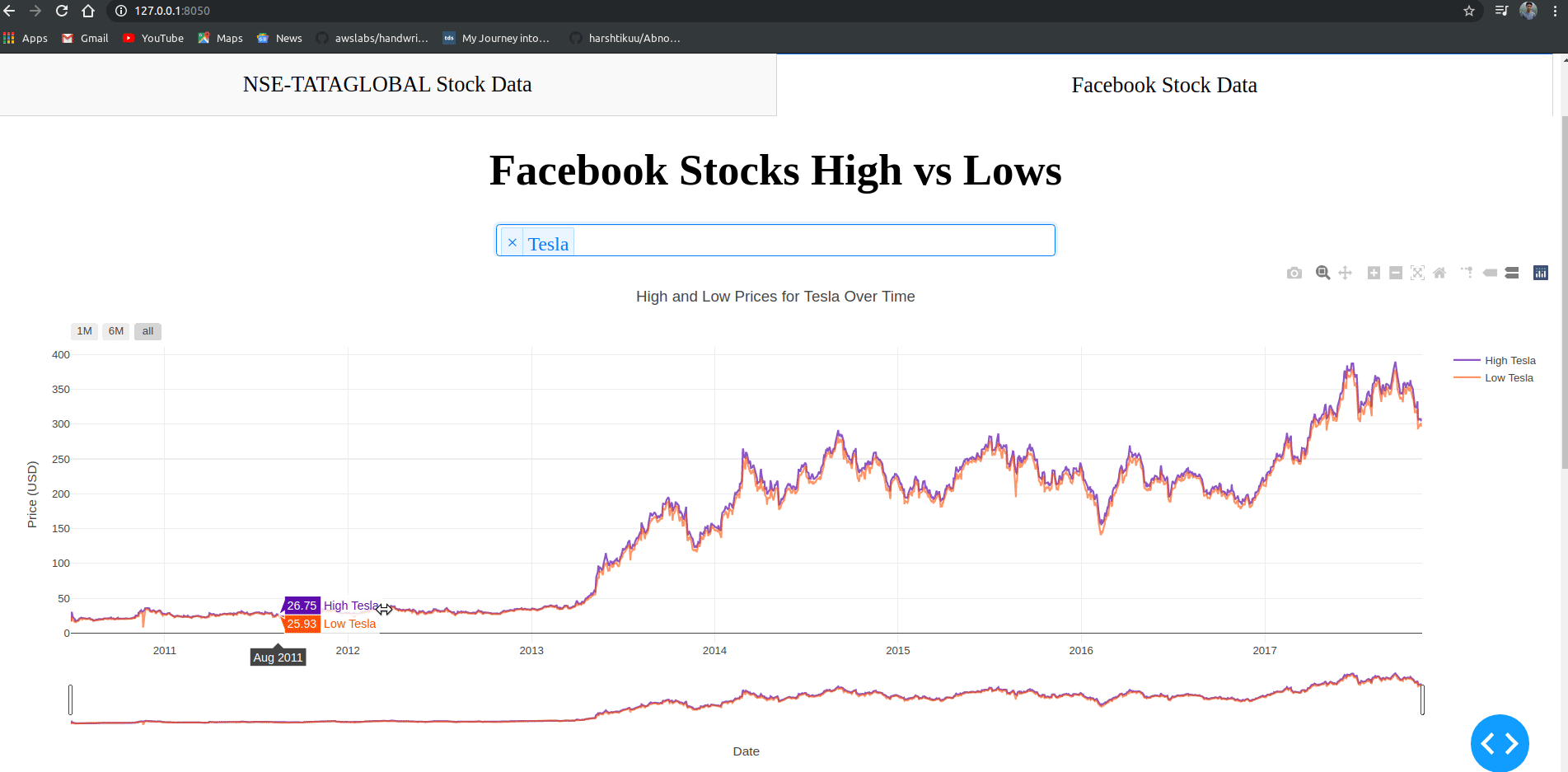# 二、分析数据

## 1.导入

import pandas as pd
import numpy as np

import matplotlib.pyplot as plt
%matplotlib inline

from matplotlib.pylab import rcParams
rcParams['figure.figsize']=20,10
from keras.models import Sequential
from keras.layers import LSTM,Dropout,Dense

from sklearn.preprocessing import MinMaxScaler


## 2.数据导入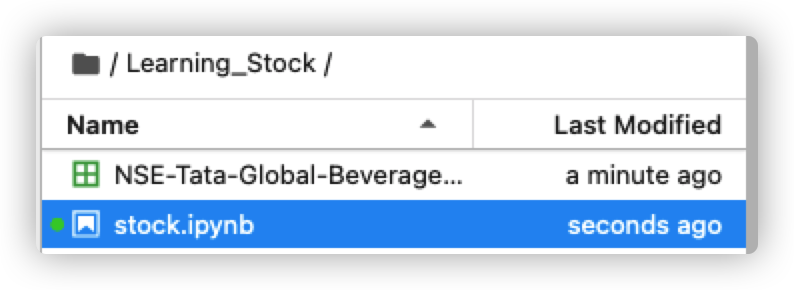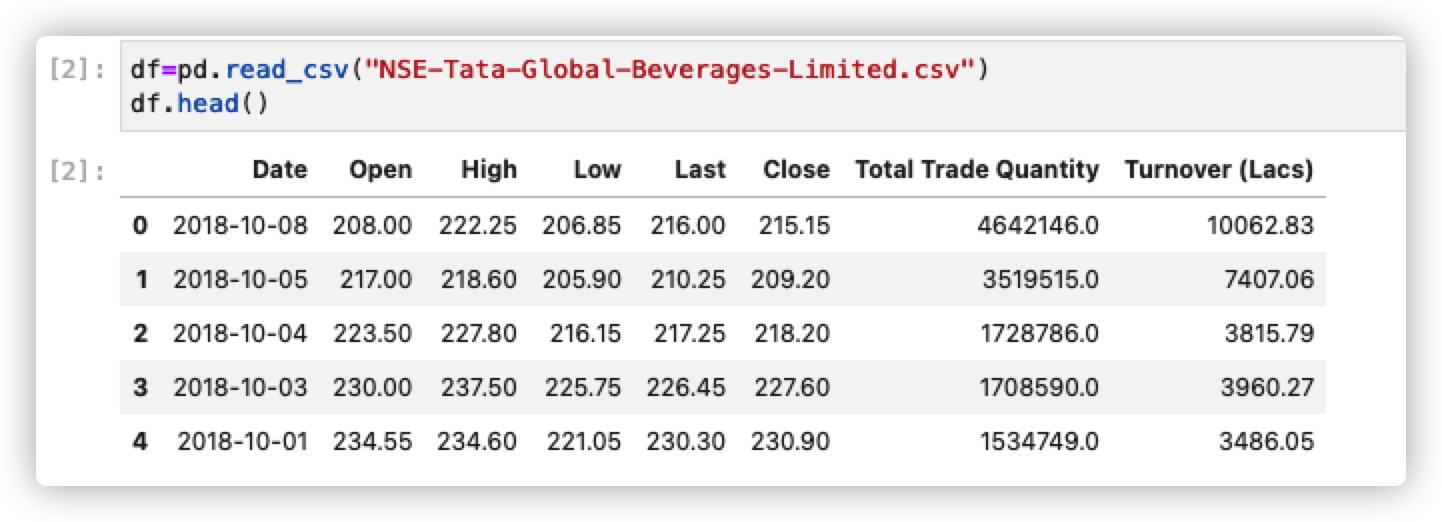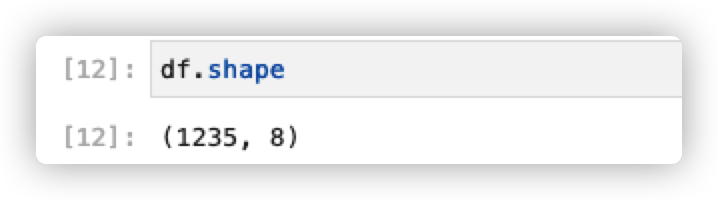## 3.分析股票尾市数据

df["Date"]=pd.to_datetime(df.Date,format="%Y-%m-%d")
df.index=df['Date']

plt.figure(figsize=(16,8))
plt.plot(df["Close"],label='Close Price history')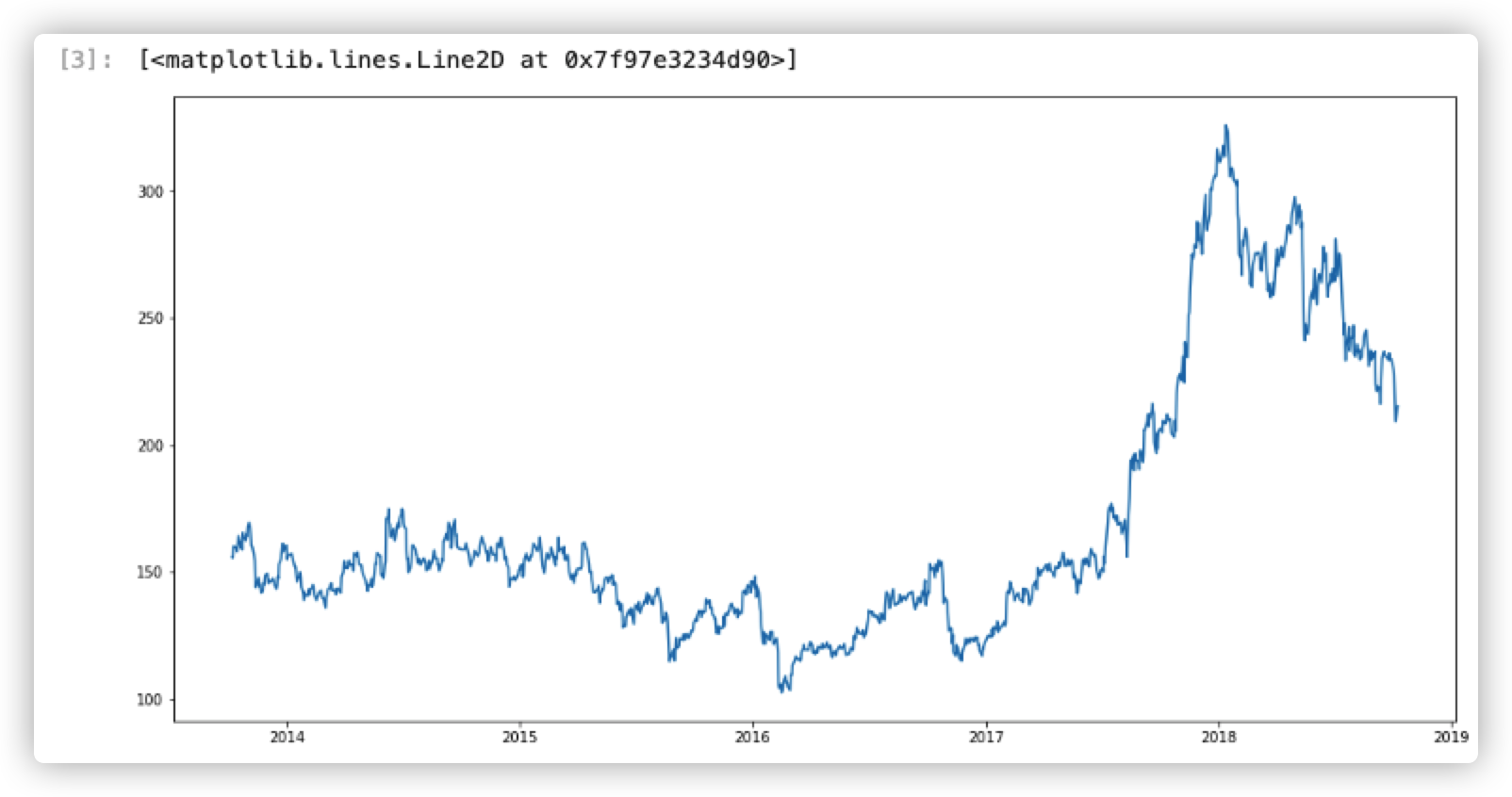## 4.构建模型

import math
#Create a new dataframe with only the Close column
data = df.filter(['Close'])
#Convert the dataframe to a numpy array
dataset = data.values

#Get the number of rows to train the model
training_data_len = math.ceil( len(dataset) * .8)
training_data_len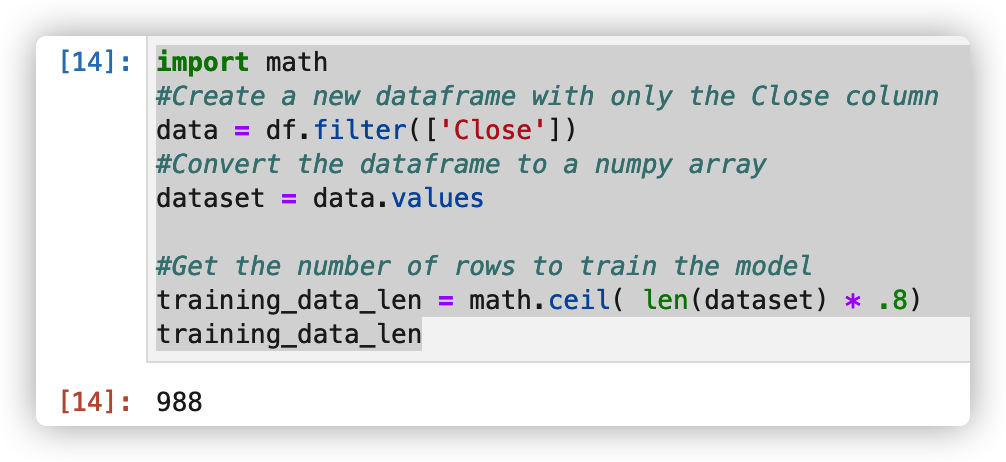#Scale the data
scaler=MinMaxScaler(feature_range=(0,1))
scaled_data=scaler.fit_transform(dataset)

scaled_data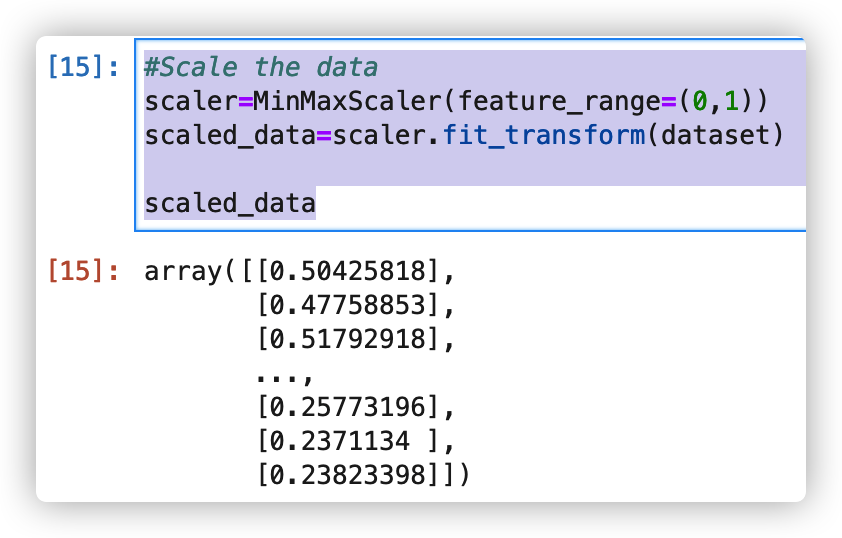#Create the training data set
#Create the scaled training data set
train_data = scaled_data[0:training_data_len , :]
#Split the data into x_train and y_train data sets
x_train = []
y_train = []

for i in range(60,len(train_data)):
x_train.append(train_data[i-60:i,0])
y_train.append(train_data[i,0])
if i<= 60:
print(x_train)
print(y_train)
print()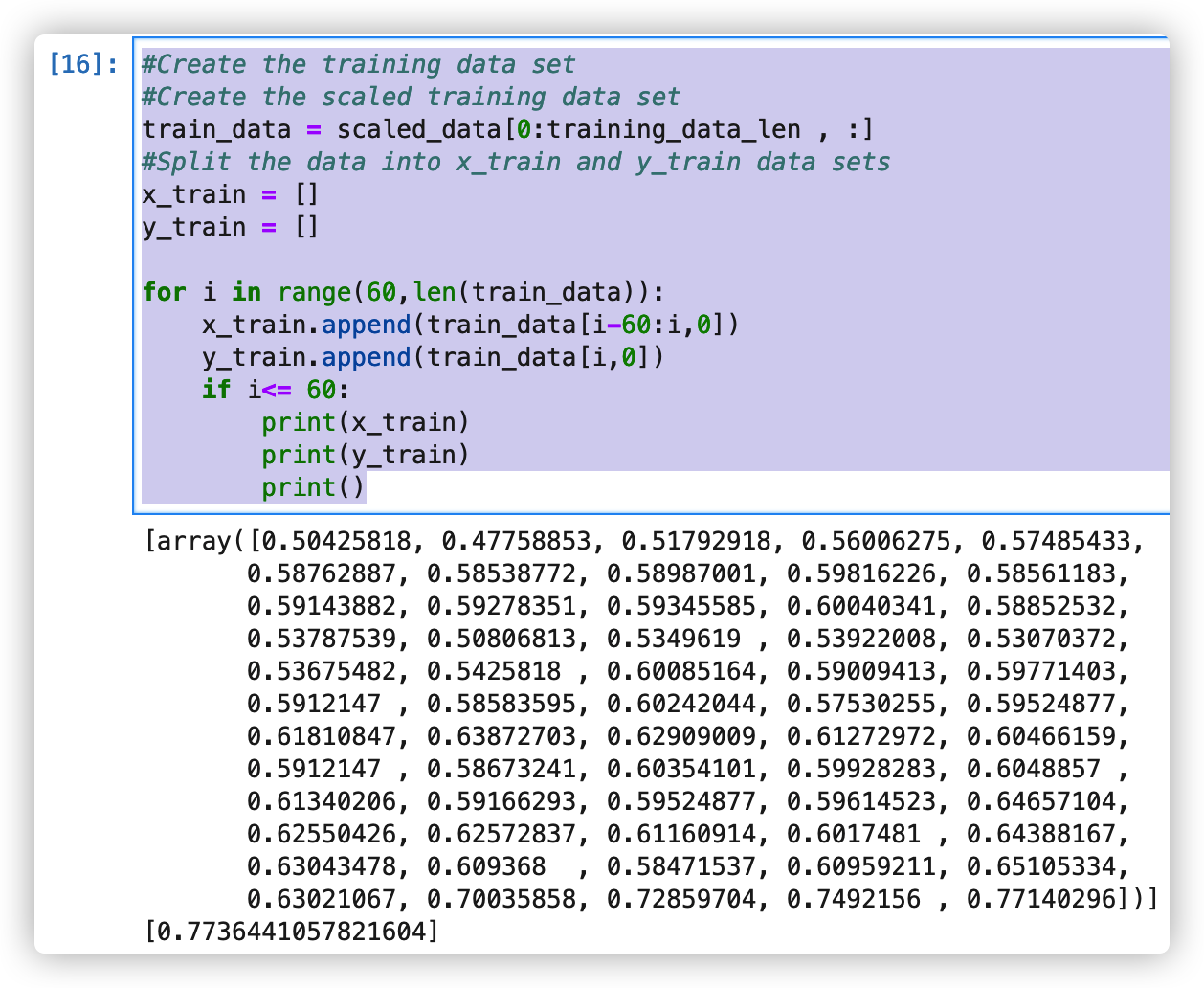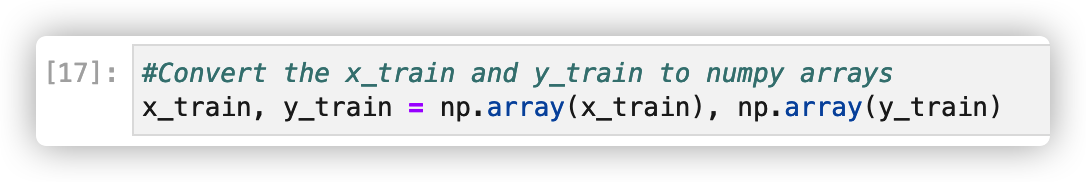#Build the LSTM model
model = Sequential()
model.add(Dense(1))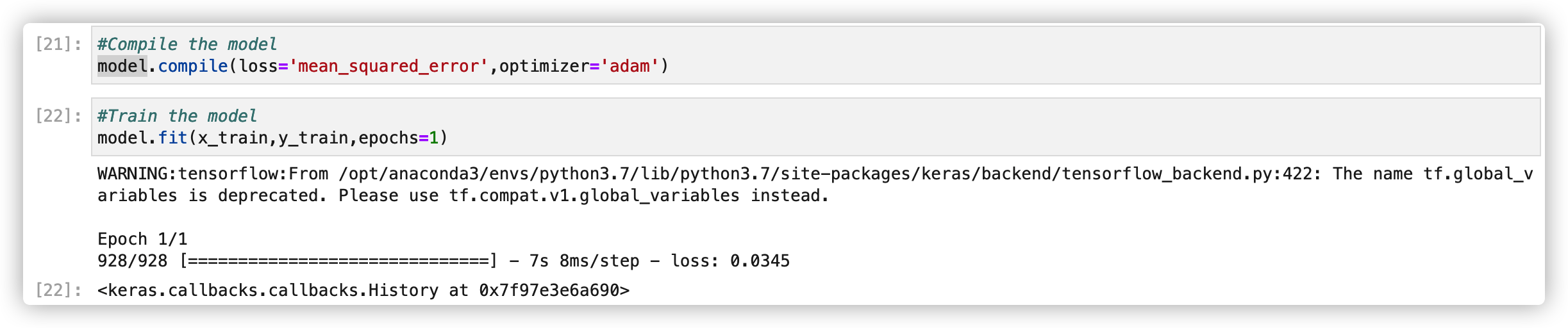## 5.测试模型

#Create the testing data set
#Create a new array containing scaled values from index 1543 to 2003
test_data = scaled_data[training_data_len - 60: , :]
#Create the data sets x_test and y_test
x_test = []
y_test = dataset[training_data_len: , :]
for i in range(60, len(test_data)):
x_test.append(test_data[i-60:i,0])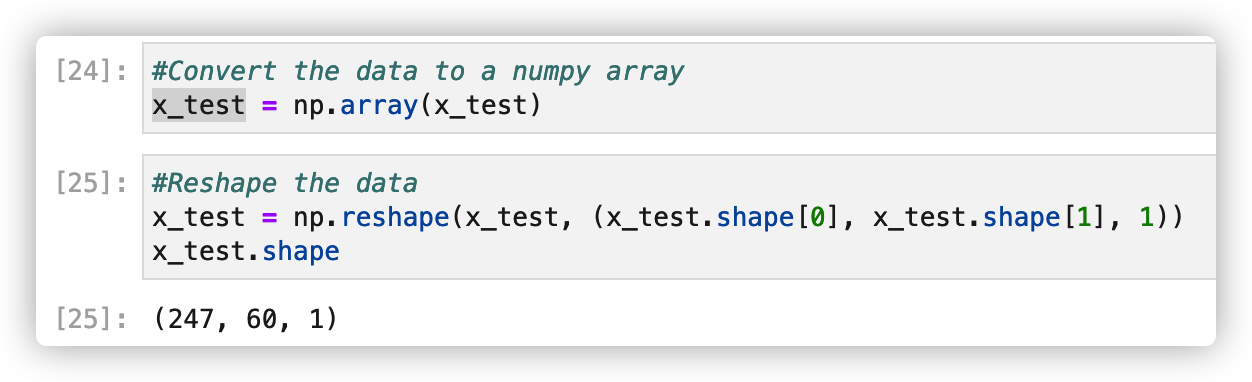#Get the models predicted price values
predictions = model.predict(x_test)
predictions = scaler.inverse_transform(predictions)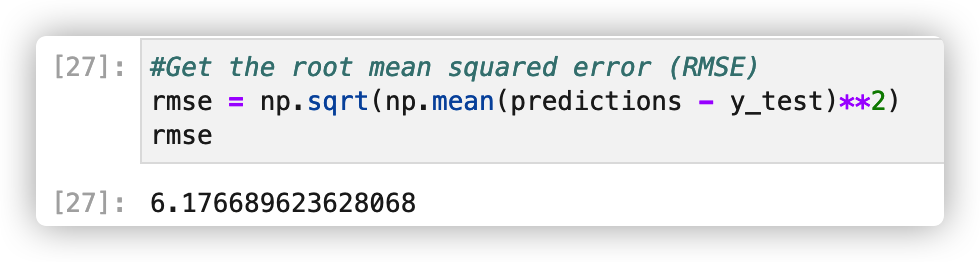## 6.展示预测结果

#Plot the data
train = data[:training_data_len]
valid = data[training_data_len:]
valid['Predictions'] = predictions
#Visualize the data
plt.figure(figsize=(16,8))
plt.title('Model')
plt.xlabel('Date', fontsize=18)
plt.ylabel('Close Prise USD (\$)', fontsize=18)
plt.plot(train['Close'])
plt.plot(valid[['Close', 'Predictions']])
plt.legend(['Train','Val','Predictions'], loc='lower right')
plt.show()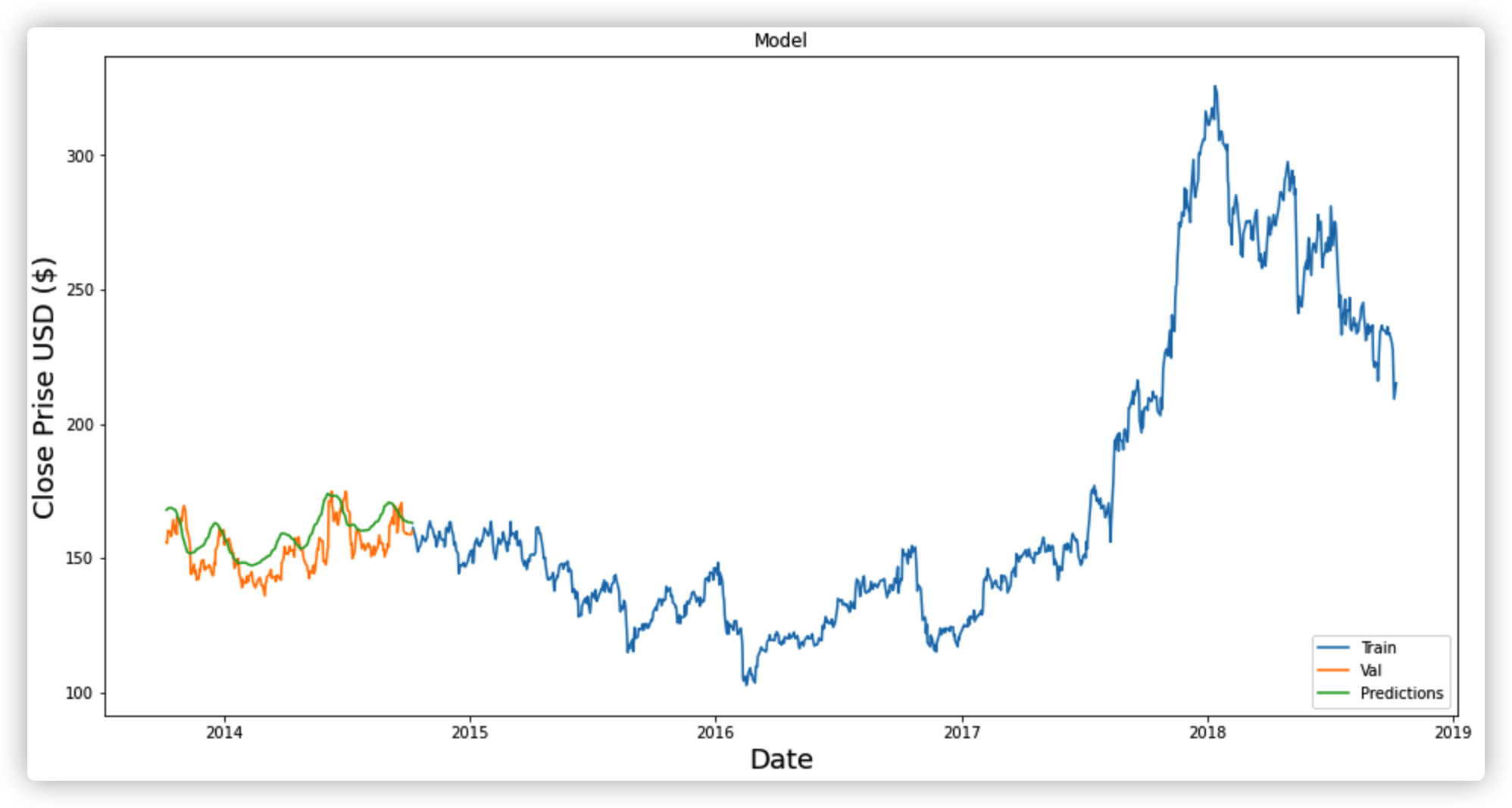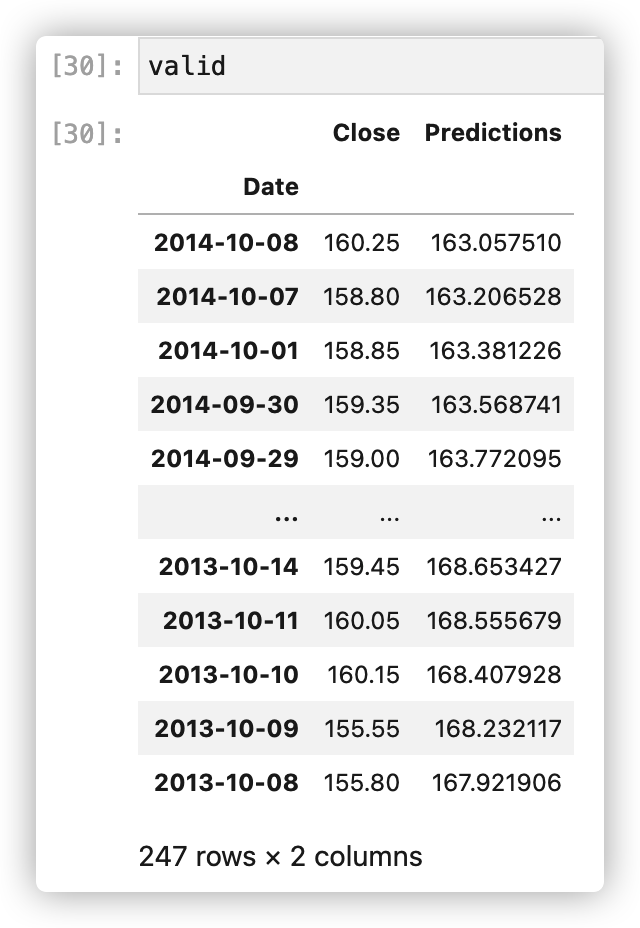展开全文股票预测
• ▼更多精彩推荐，请关注我们▼大数据文摘出品编译：Luna我们都知道，教科书上所学与实际操作还是有出入的，那关于机器学习有什么好的项目可以实操吗？文摘菌为你推荐这篇文章，在本教程中，涵盖面...人工智能 大数据 编程语言
• ## 机器学习项目实战

千人学习 2020-09-10 11:22:12
各个行业各种机器学习算法的应用实战，项目列表： 回归应用：波士顿房价预测 回归应用：葡萄酒质量和时间的关系 逻辑回归：银行用户流失预测 逻辑回归：糖尿病预测项目 KNN：鸢尾花分类 KNN：水果分类 神经网络：...机器学习 机器学习算法 机器学习实战 python机器学习
• 本课程通过一系列内容讲解和编码实战，按照由易到难，由浅入深的顺序展开，让学员在实践中，依次学习神经网络和深度学习，并且进行基于深度学习的自然语言处理技术... 建议和《机器学习项目实战》搭配使用，效果更佳~自然语言处理 深度学习 人工智能
• 机器学习总结之——机器学习项目的完整流程 1、分析项目需求，明确具体问题   进行一个项目前，首先要做的是要分析项目各个需求，以及明确各个需求所对应的具体问题并将其抽象成一个个数学问题，将项目过程...机械学习
• ↑↑↑关注后"星标"Datawhale每日干货&每月组队学习，不错过Datawhale干货来源：大数据文摘，整理：数据派THU本文约5300字，建议阅读1...大数据 人工智能 编程语言 数据挖掘
• 2020年度最佳的23个的机器学习项目（附源代码） 大数据文摘出品 编译：Luna 我们都知道，教科书上所学与实际操作还是有出入的，那关于机器学习有什么好的项目可以实操吗？ 文摘菌为你推荐这篇文章，在本...深度学习 数据挖掘 自然语言处理
• 机器学习的第一步：明确问题。 这里的抽象成数学问题，指的是明确我们可以获得什么样的数据，需要获得什么样的数据，明确目标是分类、回归、聚类等问题类型。 2 获取数据 机器学习结果的上限由数据决定，而算法...
• “ 阅读本文大概需要 4 分钟。 ”大家好，我是 Chris，入行前 5 年在一家上市游戏公司做算法，从数据挖掘算法在业务线落地开始，涉及机器学习、深度学习，后来逐步负责...
• GitHub 是计算机科学领域最为活跃...作为一名机器学习爱好者，作者在本文中列出了 2017 年 GitHub 平台上最为热门的知识库，其中包含了学习资料与工具。希望对你的学习和研究有所帮助。 目录 1. 学习资源 1. Agithub processing 人工智能 深度学习
• 这是一篇完全手把手进行机器学习项目构建的教程，包含：1. 数据清理和格式化 2. 探索性数据分析 3. 特征工程和特征选择 4. 在性能指标上比较几种机器学习模型5. 对...
• 机器学习开源项目的简单介绍 摘要: 2016 和 2017 年对机器学习来说都是令人兴奋的，机器学习领域在 2016 年取得了重大的进展，2017 年，热度继续上升......2016 年，似乎所有巨头公司都在做机器学习，如果没有，也...
• 我在原文提供的实例基础上，结合自己的分析，模拟了一个机器学习项目的分析和实现过程，包括项目分析，数据分析，模型分析，性能分析等，在原文的样本代码基础上，编写了完整的可全面自动化运行的代码，供大家一起...Python
• 在本指南中，我们将为初学者提供8个有趣的机器学习项目。项目是您当时最好的投资之一。您将享受学习，保持动力并加快进度。 你看，没有多少理论可以取代动手实践。教科书和课程可以让你陷入错误的掌握信念，因为...机器学习 入门
• Python开源机器学习项目实战 文章详细总结了当前比较火热的机器学习和深度学习的实战项目，希望能对各位人工智能爱好者有所帮助，转载请注明本博客地址。 1、Scikit-learn是一种基于 NumPy、SciPy 和matplotlib ...python 人工智能 深度学习
• ## 小白必看的经典机器学习入门项目

千次阅读 多人点赞 2021-06-10 07:20:29
在本文中，我将带您了解一个关于使用机器学习 Python 进行股票价格预测的简单数据科学项目。 在本文的最后，您将学习如何通过实现 Python 编程语言使用线性回归模型来预测股票价格。 自股市诞生以来，对其进行预测...
• 1. 实际问题抽象成数学问题这里的抽象成数学问题，指的我们明确我们可以...机器学习比赛中原始数据都是直接提供的，但是实际问题需要自己获得原始数据。“ 数据决定机器学习结果的上限，而算法只是尽可能的逼近这个上限特征工程
• 作者：Matthew Mayo, KDnuggets翻译：笪洁琼，和中华校对：丁楠雅本文共2299字，建议阅读6分钟。本文将给大家介绍五个十分可怕但还鲜为人知的机器学习项...
• 机器学习项目中，可用那么多算法，那么多Feature，模型有那么多参数，怎么选择合适的？凭直觉？算法 数据
• 解释机器学习模型并展示结果 机器学习模型经常被批评为黑盒子：我们将数据放在一边，并...我们将讨论机器学习项目中最重要的部分：记录我们的工作并展示结果。 该系列的第一部分包括数据清理，探索性数据分析，特...机器学习
• 摘要： 你是一名希望开始或者正在学习机器学习的Java程序员吗？ 利用机器学习编写程序是最佳的学习方式。你可以从头开始编写算法，但是利用现有的开源库，你可以取得更大的进步。 本文介绍了主要的平台和开放源码的...java 神经网络 人工智能
• 开源是技术创新和快速发展的核心。这篇文章向你展示Python机器学习开源项目以及在分析过程中发现的非常有趣的见解和趋势。我们分析了GitHub上的前20名Python机器...
• ## 42个机器学习练手项目

万次阅读 多人点赞 2019-12-15 10:49:43
从个性化推送（今日头条）、自拍美颜、自动翻译，到...今天，我们带来了 44 个机器学习的练手项目，分为「入门知识」、「基础课程」、「基础应用」、「综合应用」、「系统进阶」5个模块，层层递进，帮助你系统地入门...
• 我的CSDN博客地址：红色石头的专栏 ... 我的微信公众号：红色石头的机器学习之路（ID：redstonewill） 欢迎大家关注我！共同学习，共同进步！ 《Structuring Machine Learning Projects》（构建机...深度学习 吴恩达
• 一、完整机器学习项目流程 数学抽象--任务目标 明确问题是进行机器学习的第一步。机器学习的训练过程通常都是一件非常耗时的事情，胡乱尝试时间成本是非常高的。 这里的抽象成数学问题，指的是根据数据明确...
• 机器学习实战项目，适合初学的50个项目实战，均有项目介绍，资源链接，源码链接等等信息...